<![CDATA[ZNing源创库]]> 2020-07-19T17:21:11+08:00 https://zning.me/ MWeb <![CDATA[解决Elasticsearch SQL命令行启动报错 ./x-pack-env: No such file or directory]]> 2020-07-19T16:54:46+08:00 https://zning.me/15951488866193.html 今天在云服务器上，通过下载源码包完成有认证配置的Elasticsearch部署后，准备执行如下命令进入Elasticsearch SQL进行一些SQL语句的操作：

bin/elasticsearch-sql-cli uri=http://elastic:ESabc+2333@10.66.66.2:9200/


#!/bin/bash

# you may not use this file except in compliance with the Elastic License.

source "dirname "$0""/elasticsearch-env source "dirname "$0""/x-pack-env

CLI_JAR=$(ls$ES_HOME/bin/elasticsearch-sql-cli-*.jar)

exec \
"$JAVA" \ -jar "$CLI_JAR" \
"$@"  其中有效行第二行的source "dirname "$0""/x-pack-env看来执行起来有问题，估计是在依赖导入生效时找不到路径。经过一番折腾，解决了该问题，即将该行替换为如下命令：

# source "dirname "$0""/x-pack-env source /usr/share/elasticsearch/bin/x-pack-env  即将引入依赖的路径写死即可，使用时请根据实际路径修改x-pack-env的指向路径。 修改完后，再用刚才的命令启动Elasticsearch SQL，正常进入，完美结局。 后来又仔细查找了下资料，发现该问题是Elasticsearch 7.4版本的一个小bug，在Elasticsearch 7.8的包内，bin/elasticsearch-sql-cli这个脚本内容已经修改为： #!/bin/bash # Copyright Elasticsearch B.V. and/or licensed to Elasticsearch B.V. under one # or more contributor license agreements. Licensed under the Elastic License; # you may not use this file except in compliance with the Elastic License. source "dirname "$0""/elasticsearch-env

source "$ES_HOME"/bin/x-pack-env CLI_JAR=$(ls "$ES_HOME"/bin/elasticsearch-sql-cli-*.jar) exec \ "$JAVA" \
"$XSHARE" \ -jar "$CLI_JAR" \
"$@"  也就是说，如果Elasticsearch 7.8安装时，操作系统环境变量内$ES_HOME能够正确设置的话，就可以正常启动Elasticsearch SQL了。这样比直接修改产品本身脚本来说要规范的多了。

## 参考文献

]]>
<![CDATA[“源产控”系列（三）在CentOS 8上使用Elastic Stack: Elasticsearch/Kibana 7.8的部署与认证配置]]> 2020-07-18T17:46:14+08:00 https://zning.me/15950655748460.html

## Elastic Stack介绍

Elastic Stack包含：

• Elasticsearch是个开源分布式搜索引擎，提供搜集、分析、存储数据三大功能。它的特点有：分布式，零配置，自动发现，索引自动分片，索引副本机制，RESTful风格接口，多数据源，自动搜索负载等。详细可参考Elasticsearch权威指南。

• Logstash主要是用来日志的搜集、分析、过滤日志的工具，支持大量的数据获取方式。一般工作方式为C/S架构，client端安装在需要收集日志的主机上，Server端负责将收到的各节点日志进行过滤、修改等操作在一并发往Elasticsearch上去。

• Kibana也是一个开源和免费的工具，Kibana可以为 Logstash 和 Elasticsearch 提供的日志分析友好的 Web 界面，可以帮助汇总、分析和搜索重要数据日志。

• Beats在这里是一个轻量级日志采集器，其实Beats家族有6个成员，早期的ELK架构中使用Logstash收集、解析日志，但是Logstash对内存、CPU、I/O等资源消耗比较高。相比 Logstash，Beats所占系统的CPU和内存几乎可以忽略不计。

## Elasticsearch 7.8的部署

### 方式一：YUM方式安装

rpm --import https://artifacts.elastic.co/GPG-KEY-elasticsearch


[elasticsearch]
name=Elasticsearch repository for 7.x packages
baseurl=https://artifacts.elastic.co/packages/7.x/yum
gpgcheck=1
gpgkey=https://artifacts.elastic.co/GPG-KEY-elasticsearch
enabled=0
autorefresh=1
type=rpm-md


### 方式二：下载RPM包手工安装

wget https://artifacts.elastic.co/downloads/elasticsearch/elasticsearch-7.8.0-x86_64.rpm;
shasum -a 512 -c elasticsearch-7.8.0-x86_64.rpm.sha512;
rpm --install elasticsearch-7.8.0-x86_64.rpm;


### NOT starting on installation, please execute the following statements to configure elasticsearch service to start automatically using systemd
sudo systemctl enable elasticsearch.service
### You can start elasticsearch service by executing
sudo systemctl start elasticsearch.service
Created elasticsearch keystore in /etc/elasticsearch/elasticsearch.keystore
[/usr/lib/tmpfiles.d/elasticsearch.conf:1] Line references path below legacy directory /var/run/, updating /var/run/elasticsearch → /run/elasticsearch; please update the tmpfiles.d/ drop-in file accordingly.


### 方式三：源码安装

groupadd -g 888 elasticsearch;
useradd -g elasticsearch -m -u 888 elasticsearch;


wget https://artifacts.elastic.co/downloads/elasticsearch/elasticsearch-7.8.0-linux-x86_64.tar.gz;
tar -zxvf elasticsearch-7.8.0-linux-x86_64.tar.gz -C /usr/share/;
mv /usr/share/elasticsearch-7.8.0/ /usr/share/elasticsearch;
chown -R elasticsearch:elasticsearch /usr/share/elasticsearch;


## Elasticsearch 7.8的配置

-Xms4g
-Xmx4g


-Xms4096m
-Xmx4096m


# 集群名称，集群中所有节点统一
cluster.name: Elasticsearch-Cluster
# 当前节点名，自定义但集群内不允许重复
node.name: node-1
node.attr.rack: r1
# 下方数据和日志存放路径请按照实际情况修改路径
# 通过方法一、二安装，默认路径已存在，通过方法三安装，请指定存在的路径
path.data: /var/lib/elasticsearch
path.logs: /var/logs/elasticsearch
bootstrap.memory_lock: true
# 当前节点内网IP地址，虽也可以设置为0.0.0.0但建议还是按此设置
network.host: 10.66.66.1
http.port: 9200
# 集群中所有节点地址
discovery.seed_hosts: ["10.66.66.1", "10.66.66.2","10.66.66.3"]
# 集群中所有节点名，应与集群所有节点的node.name一致
cluster.initial_master_nodes: ["node-1", "node-2","node-3"]


elasticsearch      soft    nofile  100000
elasticsearch      hard    nofile  100000
elasticsearch      soft    memlock unlimited
elasticsearch      hard    memlock unlimited
elasticsearch      soft    nproc   4096
elasticsearch      hard    nproc   4096


vm.swappiness=10
vm.max_map_count=262144


10.66.66.1  node-1
10.66.66.2  node-2
10.66.66.3  node-3


Elasticsearch内置Java，无需再在系统层再次部署Java。

systemctl start elasticsearch;
systemctl stop elasticsearch;
systemctl restart elasticsearch;


systemctl daemon-reload;
systemctl enable elasticsearch;


# 启动
/usr/share/elasticsearch/bin/elasticsearch -d -p /usr/share/elasticsearch/pid;
# 停止
esid=$(cat /usr/share/elasticsearch/pid && echo); kill -SIGTERM$esid;


# 路径请注意：1. 按照配置文件设置的日志存放路径寻找；2. 按照实际集群名输入日志文件名
tail -f /var/logs/elasticsearch/Elasticsearch-Cluster.log;
# 按照方法一、二安装后也可以通过如下命令查看Elasticsearch进程情况
systemctl status elasticsearch;


curl -XGET http://node-1:9200/_cluster/health?pretty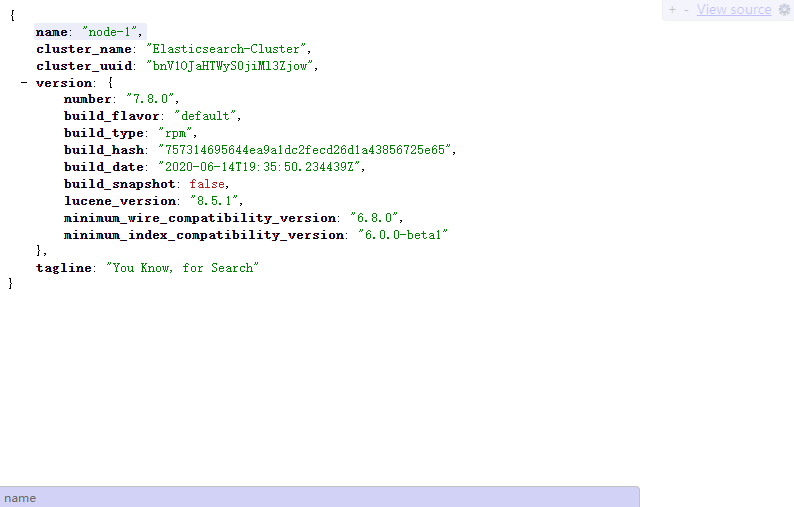## Elasticsearch 7.8的认证功能配置

Elasticsearch默认启动后，9200端口通过设定的Host IP是可以随意访问的，这十分不安全。为了保证一定的安全性，我们可以修改elasticsearch.yml文件里http.port字段，修改默认端口，以及增加访问时必须帐密认证访问。

bin/elasticsearch-certutil ca;


bin/elasticsearch-certutil cert --ca elastic-stack-ca.p12;


chown -R elasticsearch:elasticsearch *.p12;


ln -s /usr/share/elasticsearch/elastic-certificates.p12 /etc/elasticsearch/elastic-certificates.p12;
ln -s /usr/share/elasticsearch/elastic-stack-ca.p12 /etc/elasticsearch/elastic-stack-ca.p12;


# 设置集群互信通信端口9300
transport.port: 9300
http.cors.enabled: true
http.cors.allow-origin: "*"
# 开启X-Pack的安全认证
xpack.security.enabled: true
# 开启X-Pack的集群内互信安全认证，与上面安全认证开关同步必开
xpack.security.transport.ssl.enabled: true
# 验证模式为证书模式
xpack.security.transport.ssl.verification_mode: certificate
# 配置证书路径
xpack.security.transport.ssl.keystore.path: elastic-certificates.p12
xpack.security.transport.ssl.truststore.path: elastic-certificates.p12
# 如果需要启用SSL/TLS通过HTTPS访问ES集群，再添加如下配置
xpack.security.http.ssl.enabled: true
xpack.security.http.ssl.keystore.path: elastic-certificates.p12
xpack.security.http.ssl.truststore.path: elastic-certificates.p12
xpack.security.http.ssl.client_authentication: none


discovery.seed_hosts: ["10.66.66.1:9300", "10.66.66.2:9300","10.66.66.3:9300"]


bin/elasticsearch-setup-passwords interactive;


• elastic：拥有 superuser 角色，是内置的超级用户，它可以做任何事情。
• kibana：拥有 kibana_system 角色，是Kibana用来连接Elasticsearch并与之通信的。Kibana服务器以该用户身份提交请求以访问集群监视API和.kibana索引不能访问 index。
• logstash_system：拥有logstash_system角色。是Logstash在Elasticsearch中存储监控信息时使用。
• beats_system：拥有 beats_system 角色。是Beats在Elasticsearch中存储监控信息时使用。
• apm_system：APM服务器在Elasticsearch中存储监视信息时使用的用户。
• remote_monitoring_user：Metricbeat用户在Elasticsearch中收集和存储监视信息时使用。 它具有remote_monitoring_agent和remote_monitoring_collector内置角色。

curl -uelastic:ESabc+2333 -XGET http://node-1:9200/_cluster/health?pretty


## Kibana的部署与配置

Kibana的部署配置比较简单，安装方式类似Elasticsearch，具体可自行选择，本节不再赘述，使用方法二，执行如下命令，进行安装：

wget https://artifacts.elastic.co/downloads/kibana/kibana-7.8.0-x86_64.rpm;
shasum -a 512 kibana-7.8.0-x86_64.rpm;
rpm --install kibana-7.8.0-x86_64.rpm;


server.port: 5601
server.host: "0.0.0.0"
# 设置Elasticsearch集群地址，方便Kibana做容灾管理
elasticsearch.hosts: ["https://node-1:9200","https://node-2:9200","https://node-3:9200"]
kibana.index: ".kibana"
# 国际化设置，设置为中文
i18n.locale: "zh-CN"
# 开启X-Pack的安全认证
xpack.security.enabled: true
# Elasticsearch内置账户密码
# Kibana SSL/TLS访问开启，若无需配置SSL/TLS，可忽略
server.ssl.enabled: true
server.ssl.key: /etc/kibana/kibana-certificates.key
server.ssl.certificate: /etc/kibana/kibana-certificates.cer
server.ssl.certificateAuthorities: /etc/kibana/kibana-certificates-ca.cer
server.ssl.clientAuthentication: none
# Elasticsearch如开启SSL/TLS访问，则需要配置如下两条规则
elasticsearch.ssl.verificationMode: certificate
elasticsearch.ssl.certificateAuthorities: /etc/kibana/kibana-certificates-ca.cer


openssl pkcs12 -in elastic-certificates.p12 -nocerts -nodes > kibana-certificates.key
openssl pkcs12 -in elastic-certificates.p12 -clcerts -nokeys > kibana-certificates.cer
openssl pkcs12 -in elastic-certificates.p12 -cacerts -nokeys -chain > kibana-certificates-ca.cer


systemctl start kibana;
systemctl stop kibana;
systemctl restart kibana;


systemctl daemon-reload;
systemctl enable kibana;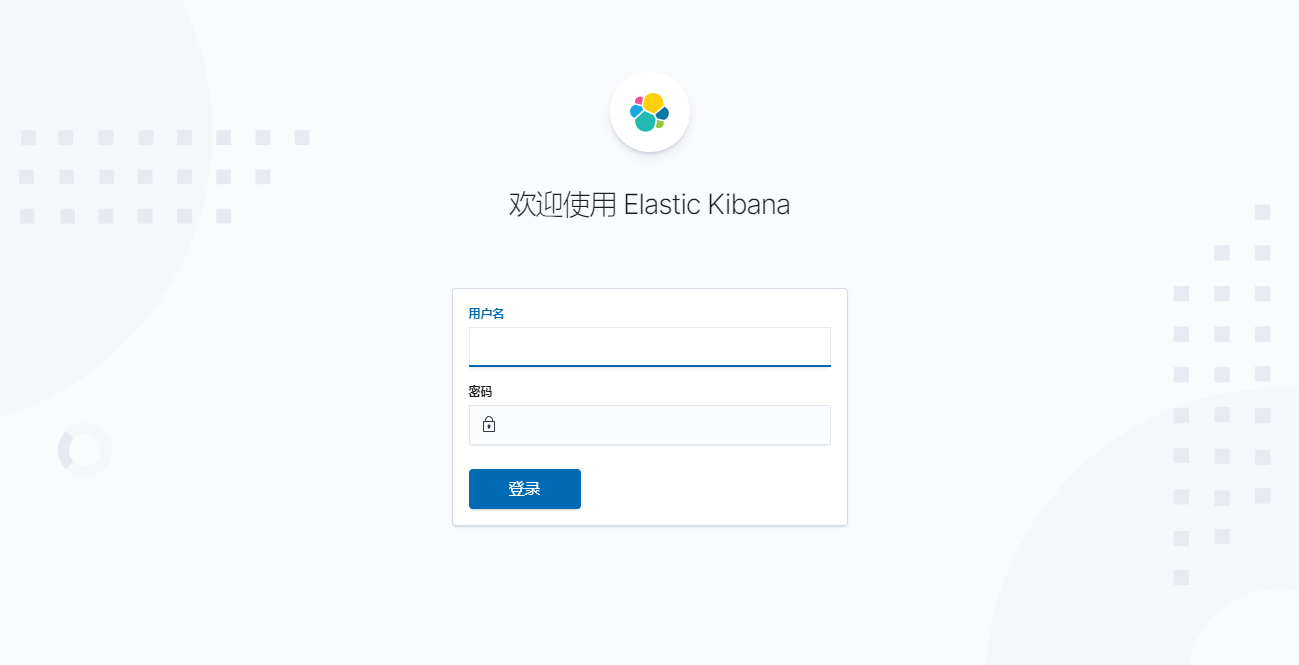]]>
<![CDATA[解决CentOS在执行yum命令时报错"Error: rpmdb open failed"]]> 2020-07-13T21:15:55+08:00 https://zning.me/15946461550517.html 今天在日常进行CentOS操作的时候，不知为何yum -y install xxx命令突然卡死不动，Ctrl+C取消执行也无解，遂关闭SSH重新建立连接，然而再次重新执行yum -y install xxx命令时却报如下错误：

...
root@localhost:~>yum -y install xxx
Existing lock /var/run/yum.pid: another copy is running as pid 27970.
Another app is currently holding the yum lock; waiting for it to exit...
The other application is: yum
Memory :  43 M RSS (362 MB VSZ)
Started: Mon Jul 13 18:27:38 2020 - 03:46 ago
State  : Uninterruptible, pid: 27970
Another app is currently holding the yum lock; waiting for it to exit...
The other application is: yum
Memory :  43 M RSS (362 MB VSZ)
Started: Mon Jul 13 18:27:38 2020 - 03:48 ago
State  : Uninterruptible, pid: 27970
...


...
root@localhost:~>yum -y install xxx
error: rpmdb: BDB0113 Thread/process 27970/140274709284672 failed: BDB1507 Thread died in Berkeley DB library
error: db5 error(-30973) from dbenv->failchk: BDB0087 DB_RUNRECOVERY: Fatal error, run database recovery
error: cannot open Packages index using db5 -  (-30973)
error: cannot open Packages database in /var/lib/rpm
CRITICAL:yum.main:

Error: rpmdb open failed
...


mv /var/lib/rpm/__db* /tmp;


rpm --rebuilddb;
yum clean all


]]>
<![CDATA[《架构即未来》之可扩展性组织的人员配置（下一）]]> 2020-07-12T19:12:23+08:00 https://zning.me/15945523435332.html 从去年夏末至今，我一直在阅读《架构即未来：现代企业可扩展的Web架构、流程和组织（原书第二版）》这本书。全书阐述了经过验证的信息技术扩展方法，对需要掌握的产品和服务的平滑扩展做了详尽的论述。具有一定的参考价值。从今天起，我将逐步总结之前所看内容，以飨读者，也以便自己回顾。文章既有摘转录，又有自我理解批注。

• 项目和任务管理是成功管理的关键。
• 人力资源管理与组织及其雇用、解雇、培养和发展相关。
• 度量是管理成功的关键。
• 管理者需要帮助团队完成任务。

## 什么是管理

《韦氏词典》中对管理是这样定义的，“执行或者监督某些事情”或“以执法手段完成某件事情”。对这一定义，我们将略加修改，融入道德因素，更新后的定义是“以明智和合乎道德的手段来完成任务”。

## 为成功铺路

]]>
<![CDATA[“源产控”系列（二）在CentOS 8上使用Nginx 1.18: 编译安装与基本使用]]> 2020-07-07T18:10:30+08:00 https://zning.me/15941166309027.html

## Nginx是个啥

Nginx（发音同“engine X”）是异步框架的网页服务器，也可以用作反向代理、负载平衡器和HTTP缓存。该软件由伊戈尔·赛索耶夫创建并于2004年首次公开发布。2011年成立同名公司以提供支持。2019年3月11日，Nginx公司被F5 Networks以6.7亿美元收购。Nginx是免费的开源软件，根据类BSD许可证的条款发布。一大部分Web服务器使用Nginx，通常作为负载均衡器。

## 在CentOS 8进行安装Nginx 1.18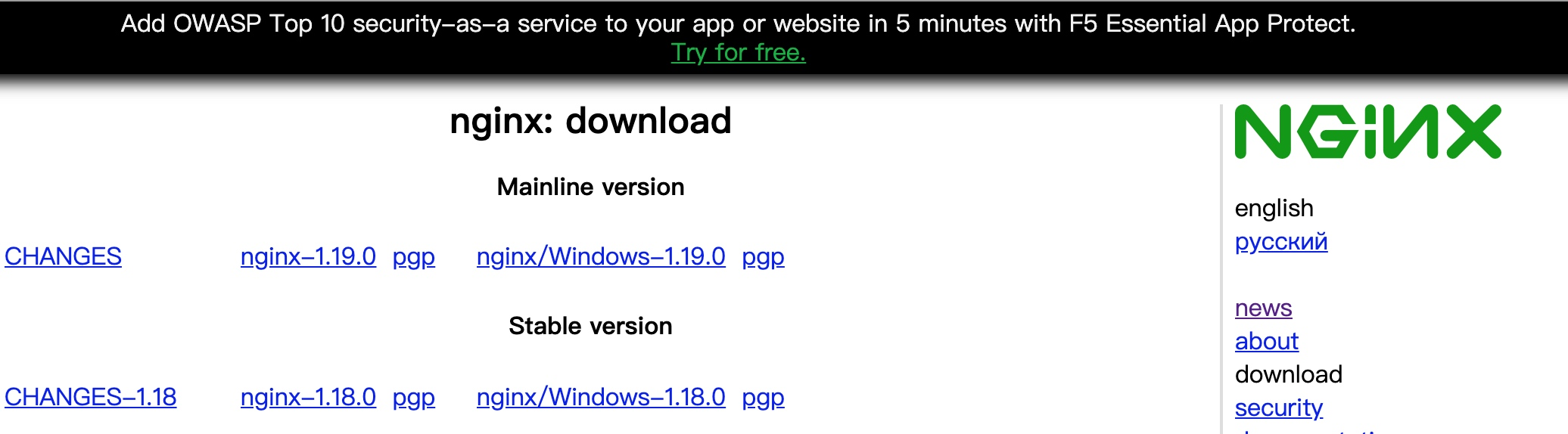Nginx版本下载页，截至发文（2020-07-07）稳定版本已更新到了1.18，主线版本更新到了1.19

wget http://nginx.org/download/nginx-1.18.0.tar.gz;
tar -zxvf nginx-1.18.0.tar.gz;


总用量 1204
drwxr-xr-x 6 1001 1001    326 7月   7 18:50 auto
-rw-r--r-- 1 1001 1001 302863 4月  21 22:09 CHANGES
-rw-r--r-- 1 1001 1001 462213 4月  21 22:09 CHANGES.ru
drwxr-xr-x 2 1001 1001    168 7月   7 18:50 conf
-rwxr-xr-x 1 1001 1001   2502 4月  21 22:09 configure
drwxr-xr-x 4 1001 1001     72 7月   7 18:50 contrib
drwxr-xr-x 2 1001 1001     40 7月   7 18:50 html
-rw-r--r-- 1 1001 1001   1397 4月  21 22:09 LICENSE
drwxr-xr-x 2 1001 1001     21 7月   7 18:50 man
-rw-r--r-- 1 1001 1001     49 4月  21 22:09 README
drwxr-xr-x 9 1001 1001     91 7月   7 18:50 src


conf文件夹内为Nginx的初始配置文件，contrib文件夹放置了三位开源贡献者贡献的三个插件内容，html文件夹为默认站点文件内容，src文件夹为Nginx源码。configure文件为编译的配置文件。

cp -r contrib/vim/* ~/.vim/;


./configure --help可以查看Nginx编译支持参数列表，configure文件进行设置后，会在objs文件内生成Nginx的安装所需文件，其中ngx_modules.c，该文件决定接下来编译有哪些模块需要编译进Nginx。

./configure --prefix=/usr/local/nginx;


...

./configure: error: the HTTP rewrite module requires the PCRE library.
You can either disable the module by using --without-http_rewrite_module
option, or install the PCRE library into the system, or build the PCRE library
statically from the source with nginx by using --with-pcre=<path> option.


Configuration summary
+ using system PCRE library
+ OpenSSL library is not used
+ using system zlib library

nginx path prefix: "/usr/local/nginx/"
nginx binary file: "/usr/local/nginx/sbin/nginx"
nginx modules path: "/usr/local/nginx/modules"
nginx configuration prefix: "/usr/local/nginx/conf"
nginx configuration file: "/usr/local/nginx/conf/nginx.conf"
nginx pid file: "/usr/local/nginx/logs/nginx.pid"
nginx error log file: "/usr/local/nginx/logs/error.log"
nginx http access log file: "/usr/local/nginx/logs/access.log"
nginx http client request body temporary files: "client_body_temp"
nginx http proxy temporary files: "proxy_temp"
nginx http fastcgi temporary files: "fastcgi_temp"
nginx http uwsgi temporary files: "uwsgi_temp"
nginx http scgi temporary files: "scgi_temp"


## 在CentOS 8初步使用Nginx 1.18

/usr/local/nginx/sbin/nginx;


Nginx基本指令格式为nginx -s reload，具体指令解释如下：

• nginx -?/-h为显示帮助信息；
• nginx -c为使用指定的配置文件，后跟配置文件路径；
• nginx -g为指定配置指令，后跟需要指定的配置指令；
• nginx-p为指定运行目录，后跟运行目录路径；
• nginx -s发送信号，其中后跟参数stop为立即停止服务、quit为有序停止服务；reload为重载配置文件、reopen为重新开始记录日志文件；
• nginx -t/-T测试配置文件是否有语法错误；
• nginx -v、nginx -V打印Nginx的版本信息或编译信息。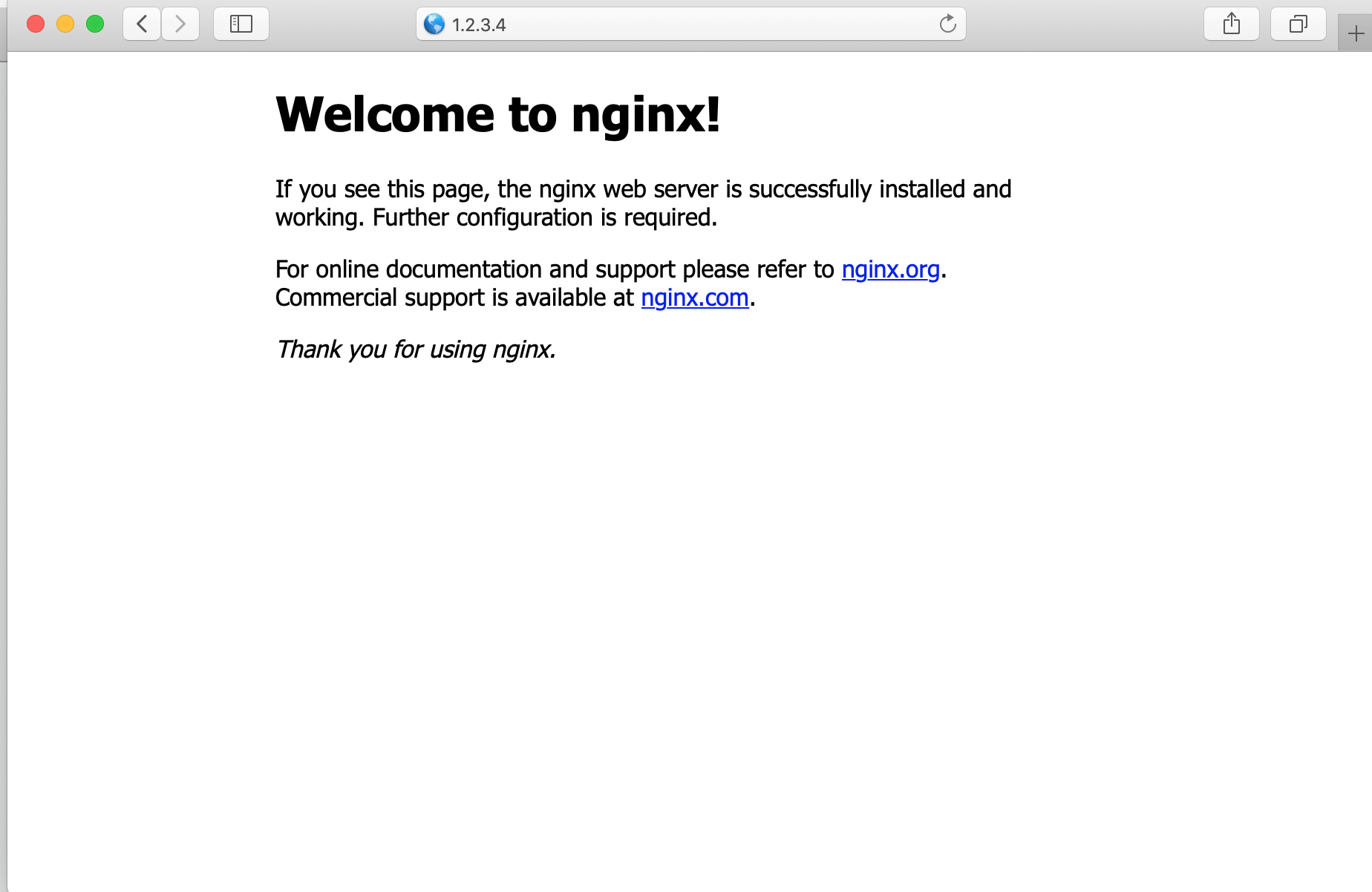ps -ef | grep nginx查看进程情况：

root      8556     1  0 19:24 ?        00:00:00 nginx: master process /usr/local/nginx/sbin/nginx
nobody    8557  8556  0 19:24 ?        00:00:00 nginx: worker process
root      8561  8702  0 19:25 pts/0    00:00:00 grep --color=auto nginx


#user  nobody;
worker_processes  auto;

error_log  logs/error.log;
#error_log  logs/error.log  notice;
#error_log  logs/error.log  info;

pid        logs/nginx.pid;

events {
worker_connections  1024;
}

http {
include       mime.types;
default_type  application/octet-stream;

log_format  main  '$remote_addr -$remote_user [$time_local] "$request" '
'$status$body_bytes_sent "$http_referer" ' '"$http_user_agent" "$http_x_forwarded_for"'; access_log logs/access.log main; server { listen 80; server_name localhost; access_log logs/host.access.log main; location / { root html; index index.html index.htm; } error_page 500 502 503 504 /50x.html; location = /50x.html { root html; } } }  Nginx配置语法有如下的规则： 1. 日志文件由指令与指令块构成； 2. 每条指令以;结尾，指令与参数间以空格符号分隔； 3. 指令块以{}将多条指令组织在一起； 4. include语句允许组合多个配置文件以提升可维护性； 5. 使用#添加注释，提高可读性； 6. 使用$可调用变量；
7. 部分指令的参数支持正则表达式。

Nginx有多种指令块，其中http块表示此为HTTP协议处理块、upstream表示有上游服务提供的配置信息、location对应URL的表达式、server对应一个或一组域/域名的访问。

• worker_processes为设定工作进程数，若设置为auto则Nginx将根据机器的内核数进行设定分配，如无特殊需求一般建议设置为auto
• error_log设定错误日志的路径与等级，其中等级有debug, info, notice, warn, error, crit, alert, emerg几种，以严重性从高到低的顺序列出。根据官方文档的解释，设定某个级别的意义是该级别及以上的日志会被打印在日志文件中，例如设置info则包括其本身在内以及notice, warn, error, crit, alert, emerg都会被列出。若第二个字段为空，默认为error
• log_format main 'XXX'设定日志格式，main标示为命名，在遇到对不同域名进行不同格式的命名记录时需要用到，server区块下access_log logs/access.log main;可以以main格式进行记录。

## 参考资料

]]>
<![CDATA[《架构即未来》之可扩展性组织的人员配置（中二）]]> 2020-07-05T19:41:43+08:00 https://zning.me/15939493033756.html 从去年夏末至今，我一直在阅读《架构即未来：现代企业可扩展的Web架构、流程和组织（原书第二版）》这本书。全书阐述了经过验证的信息技术扩展方法，对需要掌握的产品和服务的平滑扩展做了详尽的论述。具有一定的参考价值。从今天起，我将逐步总结之前所看内容，以飨读者，也以便自己回顾。文章既有摘转录，又有自我理解批注。

## 什么是领导力

【领导：天生的还是人造的】一个人的领导能力是直接取决于其影响个人或组织行为的能力。影响行为的能力来自于几个方面，有些是天生的品质，有些是环境熏陶的结果，还有些是日久形成的容易修改的工具和方法。但是经常发生的是，好的外表被当成是最重要的，但是铁的事实是大多数人都愿意围着长相好看的人转。

## 领导力概念模型

### 以人为本，使命为先

“以已为本，使命为先”的领导把员工当成向上爬的梯子，踩着他们往上走。“以人为本，使命为先”的领导则为所有的一流员工建立好向上爬的梯子。

### 愿景

• 对理想未来的生动描述
• 为股东创造价值很重要
• 可度量
• 激动人心
• 结合信仰的因素
• 大致不变，但可根据需要修改
• 容易记忆

### 使命

• 对当前状态和行动的描述
• 有目的感
• 可度量
• 一个大方向或一条通往愿景的道路

### 目标

• 具体(S）
• 可度量（M）
• 可达成(但是有挑战性）（A）
• 现实性(R）
• 时限性（或包含时间因素）（T）

## 成功的因果路线图

]]>
<![CDATA[“源产控”系列（一）CentOS 8之初相识]]> 2020-06-27T18:19:01+08:00 https://zning.me/15932531416990.html

## CentOS是什么

CentOS（Community Enterprise Operating System）是Linux发行版之一，它是来自于Red Hat Enterprise Linux（RHEL）依照开放源代码规定发布的源代码所编译而成。由于出自同样的源代码，因此有些要求高度稳定性的服务器以CentOS替代商业版的Red Hat Enterprise Linux使用。两者的不同，在于CentOS并不包含封闭源代码软件。CentOS 对上游代码的主要修改是为了移除不能自由使用的商标。2014年，CentOS宣布与Red Hat合作，但CentOS将会在新的委员会下继续运作，并不受RHEL的影响。

CentOS第一次接触在2014年，也就是CentOS与Red Hat宣布合作的那一年。印象比较深的也是那一年发行的CentOS 7，由于其与Red Hat合作后，将RHEL 7的一些新特性例如systemd等引入，导致与其CentOS 6一些操作差距过大，再加上新系统教程较少，在系统上折腾了很久，也因此印象深刻。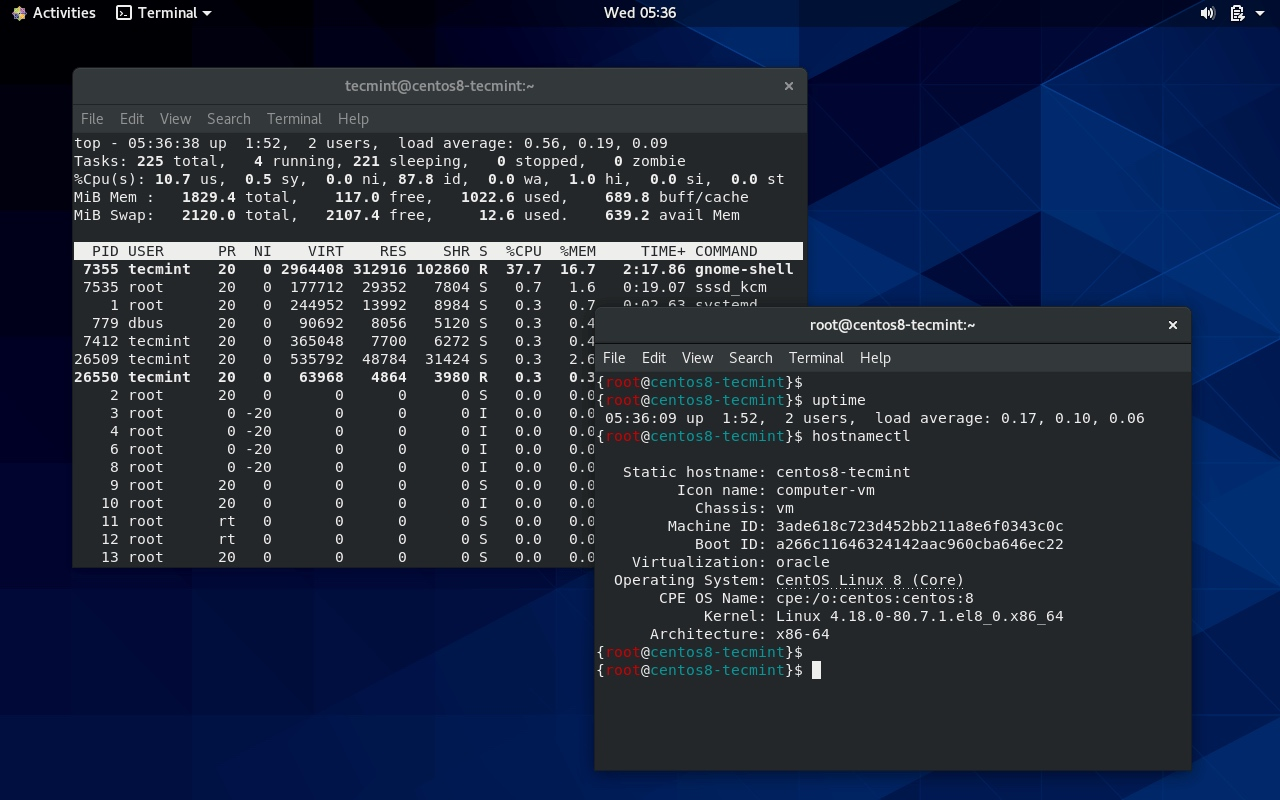## CentOS 8的新特性

CentOS项目是对Red Hat Enterprise Linux的100％兼容的重建，完全符合Red Hat的重新发布要求，并发布了一个新版本：CentOS 8.0.1905，适用于所有受支持的体系结构。

CentOS 完全遵守 Red Hat 的再发行政策，并且致力与上游产品在功能上完全兼容。CentOS 对组件的修改主要是去除 Red Hat 的商标及美工图。

CentOS 8 主要改动和 RedHat Enterprise Linux 8是一致的，基于Fedora 28和内核版本 4.18, 为用户提供一个稳定的、安全的、一致的基础，跨越混合云部署，支持传统和新兴的工作负载所需的工具。此次发布的亮点包括：

AppStream是对传统 rpm格式的全新扩展，为一个组件同时提供多个主要版本

YUM 包管理器基于 DNF 技术，提供模块化内容支持，增强了性能，并且提供了设计良好的 API 用于与其他工具集成

Shell 和命令行工具

RHEL 8 提供了版本控制工具: Git 2.18Mercurial 4.8, 和 Subversion 1.10.

Python 3.6 是默认的 Python 环境，有限支持 Python 2.7

Node.js 是在 RHEL 最新包含的，其他动态语言更新包括: PHP 7.2, Ruby 2.5, Perl 5.26, SWIG 3.0

RHEL 8 提供的数据库服务包括：MariaDB 10.3, MySQL 8.0, PostgreSQL 10, PostgreSQL 9.6, 和 Redis 5.

RHEL 8 提供Apache HTTP Server 2.4 以及首次引入的Nginx 1.14.

Squid 版本升级到 4.4 ，同时也首次提供Varnish Cache 6.0.

GNOME Shell 升级到 3.28.

GNOME 会话和显示管理使用 Wayland 作为默认的显示服务器，而 RHEL 7 默认的 X.Org server 依然提供

Anaconda 安装程序可使用 LUKS2 磁盘加密，支持 NVDIMM 设备.

Image Builder 工具可以创建不同格式的自定义系统镜像，包括满足云平台的各种格式

BPF Compiler Collection (BCC), 这是一个用来创建高效内核跟踪和操作的工具，目前处于技术预览阶段

LUKS version 2 (LUKS2) 格式替代旧的 LUKS (LUKS1) 格式. dm-crypt 子系统和 cryptsetup 工具现在使用 LUKS2 作为默认的加密卷格式

nftables 框架替代 iptables 作为默认的网络包过滤工具

firewalld 守护进程使用 nftables 作为默认后端

eXpress Data Path (XDP), XDP for Traffic Control (tc), 以及 Address Family eXpress Data Path (AF_XDP), 可作为部分 Berkeley Packet Filtering (eBPF) 扩展特性，目前还是技术预览阶段.

qemu仿真器引入了沙箱功能，它为系统调用qemu可以执行的操作提供了可配置的限制，从而使虚拟机更加安全。

GCC 编译器更新到 8.2 版本，支持更多 C++标准，更好的优化以及代码增强技术、提升警告和硬件特性支持

glibc 库升级到 2.28 支持 Unicode 11, 更新的 Linux 系统调用，关键提升主要在 DNS stub resolver 、额外的安全加强和性能提升

RHEL 8 提供 OpenJDK 11OpenJDK 8,<span> </span>IcedTea-Web, 以及不同 Java 工具，如 AntMaven, 或 Scala.

Pacemaker 集群资源管理器更新到最新版本 2.0.0, 修复了一系列 bug 以及功能做了提升

pcs 配置系统完全支持 Corosync 3, knet, 和节点名称

CentOS 8 官方发行说明：

https://lists.centos.org/pipermail/centos-announce/2019-September/023449.html

https://access.redhat.com/documentation/en-us/red_hat_enterprise_linux/8/html/8.0_release_notes/overview

]]>
<![CDATA[记一次OJ测试赛算法实现思路]]> 2020-06-10T22:53:34+08:00 https://zning.me/15918008140272.html 今下午一个小时三道题的OJ测试赛告一段落。首先一首《凉凉》送给自己。整天工作CRUD以及近期代码几乎没碰，再加上OJ刷题的日子已经过去五年了，导致犯了好几个傻瓜的基础错误，浪费了原本就宝贵的时间。题二原本估计能拿一半分的（如果后台样例形式在我估计之中的话）。

## 题三：树

]]>
<![CDATA[关于更换Maven阿里云镜像的经验总结]]> 2020-05-26T23:41:55+08:00 https://zning.me/15905077159608.html 今天需要编译flink-1.9.0flink-shaded，由于网上所给信息是，在编译时需要在两个工程内设置非中央仓库的官方镜像源（flink-1.9.0已经自带）。

<profile>
<id>vendor-repos</id>
<activation>
<property>
<name>vendor-repos</name>
</property>
</activation>
<!-- Add vendor maven repositories -->
<repositories>
<!-- Cloudera -->
<repository>
<id>cloudera-releases</id>
<url>https://repository.cloudera.com/artifactory/cloudera-repos</url>
<releases>
<enabled>true</enabled>
</releases>
<snapshots>
<enabled>false</enabled>
</snapshots>
</repository>
<!-- Hortonworks -->
<repository>
<id>HDPReleases</id>
<name>HDP Releases</name>
<url>https://repo.hortonworks.com/content/repositories/releases/</url>
<snapshots>
<enabled>false</enabled>
</snapshots>
<releases>
<enabled>true</enabled>
</releases>
</repository>
<repository>
<name>HDP Jetty</name>
<snapshots>
<enabled>false</enabled>
</snapshots>
<releases>
<enabled>true</enabled>
</releases>
</repository>
<!-- MapR -->
<repository>
<id>mapr-releases</id>
<url>https://repository.mapr.com/maven/</url>
<snapshots>
<enabled>false</enabled>
</snapshots>
<releases>
<enabled>true</enabled>
</releases>
</repository>
</repositories>
</profile>


<mirrors>
<mirror>
<id>aliyunmaven</id>
<mirrorOf>*</mirrorOf>
<name>阿里云公共仓库</name>
<url>https://maven.aliyun.com/repository/public</url>
</mirror>
<mirror>
<id>aliyunmaven</id>
<mirrorOf>*</mirrorOf>
<name>阿里云谷歌仓库</name>
</mirror>
<mirror>
<id>aliyunmaven</id>
<mirrorOf>*</mirrorOf>
<name>阿里云阿帕奇仓库</name>
<url>https://maven.aliyun.com/repository/apache-snapshots</url>
</mirror>
<mirror>
<id>aliyunmaven</id>
<mirrorOf>*</mirrorOf>
<name>阿里云spring仓库</name>
<url>https://maven.aliyun.com/repository/spring</url>
</mirror>
<mirror>
<id>aliyunmaven</id>
<mirrorOf>*</mirrorOf>
<name>阿里云spring插件仓库</name>
<url>https://maven.aliyun.com/repository/spring-plugin</url>
</mirror>
</mirrors>


<mirrors>
<mirror>
<id>aliyunmaven</id>
<mirrorOf>central</mirrorOf>
<name>aliyun maven</name>
<url>https://maven.aliyun.com/repository/public </url>
</mirror>
</mirrors>


• 若设置了<mirrorOf>*</mirrorOf>这个标签，则可能会导致工程内pom.xml相应设置的仓库失效。全局设置覆盖了工程设置。
• 应避免直接设置<mirrorOf>*</mirrorOf>这个标签，而是设置指定仓库例如<mirrorOf>central</mirrorOf>表示此为中央仓库的代理。
]]>
<![CDATA[《动手学深度学习》PyTorch版学习记录（三）]]> 2020-02-18T21:19:58+08:00 https://zning.me/15820319987185.html 近期参加了伯禹教育、Datawhale、和鲸科技组织的“《动手学深度学习》代码讲解PyTorch版”课程学习，本文为第一次学习记录。涉及知识点：过拟合、欠拟合的解决方案；梯度消失与梯度爆炸；循环神经网络。

## 过拟合、欠拟合的解决方案之权重衰减

### 方法

$$L_2$$范数正则化在模型原损失函数基础上添加$$L_2$$范数惩罚项，从而得到训练所需要最小化的函数。$$L_2$$范数惩罚项指的是模型权重参数每个元素的平方和与一个正的常数的乘积。以“线性回归”一节中的线性回归损失函数

$\ell(w_1, w_2, b) = \frac{1}{n} \sum_{i=1}^n \frac{1}{2}\left(x_1^{(i)} w_1 + x_2^{(i)} w_2 + b - y^{(i)}\right)^2$

$\ell(w_1, w_2, b) + \frac{\lambda}{2n} \|\boldsymbol{w}\|^2,$

\begin{aligned} w_1 &\leftarrow \left(1- \frac{\eta\lambda}{|\mathcal{B}|} \right)w_1 - \frac{\eta}{|\mathcal{B}|} \sum_{i \in \mathcal{B}}x_1^{(i)} \left(x_1^{(i)} w_1 + x_2^{(i)} w_2 + b - y^{(i)}\right),\\ w_2 &\leftarrow \left(1- \frac{\eta\lambda}{|\mathcal{B}|} \right)w_2 - \frac{\eta}{|\mathcal{B}|} \sum_{i \in \mathcal{B}}x_2^{(i)} \left(x_1^{(i)} w_1 + x_2^{(i)} w_2 + b - y^{(i)}\right). \end{aligned}

### 高维线性回归实验

$y = 0.05 + \sum_{i = 1}^p 0.01x_i + \epsilon,$

%matplotlib inline
import d2lzh as d2l
from mxnet import autograd, gluon, init, nd
from mxnet.gluon import data as gdata, loss as gloss, nn

n_train, n_test, num_inputs = 20, 100, 200
true_w, true_b = nd.ones((num_inputs, 1)) * 0.01, 0.05

features = nd.random.normal(shape=(n_train + n_test, num_inputs))
labels = nd.dot(features, true_w) + true_b
labels += nd.random.normal(scale=0.01, shape=labels.shape)
train_features, test_features = features[:n_train, :], features[n_train:, :]
train_labels, test_labels = labels[:n_train], labels[n_train:]


### 从零开始实现

#### 初始化模型参数

def init_params():
w = nd.random.normal(scale=1, shape=(num_inputs, 1))
b = nd.zeros(shape=(1,))
return [w, b]


#### 定义$$L_2$$范数惩罚项

def l2_penalty(w):
return (w**2).sum() / 2


#### 定义训练和测试

batch_size, num_epochs, lr = 1, 100, 0.003
net, loss = d2l.linreg, d2l.squared_loss
train_features, train_labels), batch_size, shuffle=True)

def fit_and_plot(lambd):
w, b = init_params()
train_ls, test_ls = [], []
for _ in range(num_epochs):
for X, y in train_iter:
# 添加了L2范数惩罚项
l = loss(net(X, w, b), y) + lambd * l2_penalty(w)
l.backward()
d2l.sgd([w, b], lr, batch_size)
train_ls.append(loss(net(train_features, w, b),
train_labels).mean().asscalar())
test_ls.append(loss(net(test_features, w, b),
test_labels).mean().asscalar())
d2l.semilogy(range(1, num_epochs + 1), train_ls, 'epochs', 'loss',
range(1, num_epochs + 1), test_ls, ['train', 'test'])
print('L2 norm of w:', w.norm().asscalar())


#### 观察过拟合

fit_and_plot(lambd=0)L2 norm of w: 11.611941


#### 使用权重衰减

fit_and_plot(lambd=3)L2 norm of w: 0.040959217


### 简洁实现

def fit_and_plot_gluon(wd):
net = nn.Sequential()
net.initialize(init.Normal(sigma=1))
# 对权重参数衰减。权重名称一般是以weight结尾
trainer_w = gluon.Trainer(net.collect_params('.*weight'), 'sgd',
{'learning_rate': lr, 'wd': wd})
# 不对偏差参数衰减。偏差名称一般是以bias结尾
trainer_b = gluon.Trainer(net.collect_params('.*bias'), 'sgd',
{'learning_rate': lr})
train_ls, test_ls = [], []
for _ in range(num_epochs):
for X, y in train_iter:
l = loss(net(X), y)
l.backward()
# 对两个Trainer实例分别调用step函数，从而分别更新权重和偏差
trainer_w.step(batch_size)
trainer_b.step(batch_size)
train_ls.append(loss(net(train_features),
train_labels).mean().asscalar())
test_ls.append(loss(net(test_features),
test_labels).mean().asscalar())
d2l.semilogy(range(1, num_epochs + 1), train_ls, 'epochs', 'loss',
range(1, num_epochs + 1), test_ls, ['train', 'test'])
print('L2 norm of w:', net.weight.data().norm().asscalar())


fit_and_plot_gluon(0)L2 norm of w: 13.311795

fit_and_plot_gluon(3)L2 norm of w: 0.032668564


### 小结

• 正则化通过为模型损失函数添加惩罚项使学出的模型参数值较小，是应对过拟合的常用手段。
• 权重衰减等价于$$L_2$$范数正则化，通常会使学到的权重参数的元素较接近0。
• 权重衰减可以通过Gluon的wd超参数来指定。
• 可以定义多个Trainer实例对不同的模型参数使用不同的迭代方法。

### 练习

• 回顾一下训练误差和泛化误差的关系。除了权重衰减、增大训练量以及使用复杂度合适的模型，你还能想到哪些办法来应对过拟合？
• 如果你了解贝叶斯统计，你觉得权重衰减对应贝叶斯统计里的哪个重要概念？
• 调节实验中的权重衰减超参数，观察并分析实验结果。

## 过拟合、欠拟合的解决方案之丢弃法

### 方法

$h_i = \phi\left(x_1 w_{1i} + x_2 w_{2i} + x_3 w_{3i} + x_4 w_{4i} + b_i\right),$

$h_i' = \frac{\xi_i}{1-p} h_i.$

$E(h_i') = \frac{E(\xi_i)}{1-p}h_i = h_i.$### 从零开始实现

import d2lzh as d2l
from mxnet import autograd, gluon, init, nd
from mxnet.gluon import loss as gloss, nn

def dropout(X, drop_prob):
assert 0 <= drop_prob <= 1
keep_prob = 1 - drop_prob
# 这种情况下把全部元素都丢弃
if keep_prob == 0:
return X.zeros_like()
mask = nd.random.uniform(0, 1, X.shape) < keep_prob
return mask * X / keep_prob


X = nd.arange(16).reshape((2, 8))
dropout(X, 0)

[[ 0.  1.  2.  3.  4.  5.  6.  7.]
[ 8.  9. 10. 11. 12. 13. 14. 15.]]
<NDArray 2x8 @cpu(0)>

dropout(X, 0.5)

[[ 0.  2.  4.  6.  0.  0.  0. 14.]
[ 0. 18.  0.  0. 24. 26. 28.  0.]]
<NDArray 2x8 @cpu(0)>

dropout(X, 1)

[[0. 0. 0. 0. 0. 0. 0. 0.]
[0. 0. 0. 0. 0. 0. 0. 0.]]
<NDArray 2x8 @cpu(0)>


#### 定义模型参数

num_inputs, num_outputs, num_hiddens1, num_hiddens2 = 784, 10, 256, 256

W1 = nd.random.normal(scale=0.01, shape=(num_inputs, num_hiddens1))
b1 = nd.zeros(num_hiddens1)
W2 = nd.random.normal(scale=0.01, shape=(num_hiddens1, num_hiddens2))
b2 = nd.zeros(num_hiddens2)
W3 = nd.random.normal(scale=0.01, shape=(num_hiddens2, num_outputs))
b3 = nd.zeros(num_outputs)

params = [W1, b1, W2, b2, W3, b3]
for param in params:


#### 定义模型

drop_prob1, drop_prob2 = 0.2, 0.5

def net(X):
X = X.reshape((-1, num_inputs))
H1 = (nd.dot(X, W1) + b1).relu()
H1 = dropout(H1, drop_prob1)  # 在第一层全连接后添加丢弃层
H2 = (nd.dot(H1, W2) + b2).relu()
H2 = dropout(H2, drop_prob2)  # 在第二层全连接后添加丢弃层
return nd.dot(H2, W3) + b3


#### 训练和测试模型

num_epochs, lr, batch_size = 5, 0.5, 256
loss = gloss.SoftmaxCrossEntropyLoss()
d2l.train_ch3(net, train_iter, test_iter, loss, num_epochs, batch_size,
params, lr)

epoch 1, loss 1.1250, train acc 0.564, test acc 0.773
epoch 2, loss 0.5834, train acc 0.784, test acc 0.784
epoch 3, loss 0.4944, train acc 0.820, test acc 0.847
epoch 4, loss 0.4462, train acc 0.837, test acc 0.858
epoch 5, loss 0.4169, train acc 0.848, test acc 0.867


### 简洁实现

net = nn.Sequential()
nn.Dropout(drop_prob1),  # 在第一个全连接层后添加丢弃层
nn.Dense(256, activation="relu"),
nn.Dropout(drop_prob2),  # 在第二个全连接层后添加丢弃层
nn.Dense(10))
net.initialize(init.Normal(sigma=0.01))


trainer = gluon.Trainer(net.collect_params(), 'sgd', {'learning_rate': lr})
d2l.train_ch3(net, train_iter, test_iter, loss, num_epochs, batch_size, None,
None, trainer)

epoch 1, loss 1.1132, train acc 0.565, test acc 0.782
epoch 2, loss 0.5729, train acc 0.788, test acc 0.840
epoch 3, loss 0.4903, train acc 0.821, test acc 0.847
epoch 4, loss 0.4407, train acc 0.838, test acc 0.860
epoch 5, loss 0.4163, train acc 0.847, test acc 0.839


### 小结

• 我们可以通过使用丢弃法应对过拟合。
• 丢弃法只在训练模型时使用。

### 练习

• 如果把本节中的两个丢弃概率超参数对调，会有什么结果？
• 增大迭代周期数，比较使用丢弃法与不使用丢弃法的结果。
• 如果将模型改得更加复杂，如增加隐藏层单元，使用丢弃法应对过拟合的效果是否更加明显？
• 以本节中的模型为例，比较使用丢弃法与权重衰减的效果。如果同时使用丢弃法和权重衰减，效果会如何？

### 参考文献

 Srivastava, N., Hinton, G., Krizhevsky, A., Sutskever, I., & Salakhutdinov, R. (2014). Dropout: a simple way to prevent neural networks from overfitting. JMLR

## 数值稳定性和模型初始化

### 随机初始化模型参数

#### Xavier随机初始化

$U\left(-\sqrt{\frac{6}{a+b}}, \sqrt{\frac{6}{a+b}}\right).$

### 小结

• 深度模型有关数值稳定性的典型问题是衰减和爆炸。当神经网络的层数较多时，模型的数值稳定性容易变差。
• 我们通常需要随机初始化神经网络的模型参数，如权重参数。

### 练习

• 有人说随机初始化模型参数是为了“打破对称性”。这里的“对称”应如何理解？
• 是否可以将线性回归或softmax回归中所有的权重参数都初始化为相同值？

### 参考文献

 Glorot, X., & Bengio, Y. (2010, March). Understanding the difficulty of training deep feedforward neural networks. In Proceedings of the thirteenth international conference on artificial intelligence and statistics (pp. 249-256).

## 循环神经网络

### 不含隐藏状态的神经网络

$\boldsymbol{H} = \phi(\boldsymbol{X} \boldsymbol{W}_{xh} + \boldsymbol{b}_h),$

$\boldsymbol{O} = \boldsymbol{H} \boldsymbol{W}_{hq} + \boldsymbol{b}_q,$

### 含隐藏状态的循环神经网络

$\boldsymbol{H}_t = \phi(\boldsymbol{X}_t \boldsymbol{W}_{xh} + \boldsymbol{H}_{t-1} \boldsymbol{W}_{hh} + \boldsymbol{b}_h).$

$\boldsymbol{O}_t = \boldsymbol{H}_t \boldsymbol{W}_{hq} + \boldsymbol{b}_q.$from mxnet import nd

X, W_xh = nd.random.normal(shape=(3, 1)), nd.random.normal(shape=(1, 4))
H, W_hh = nd.random.normal(shape=(3, 4)), nd.random.normal(shape=(4, 4))
nd.dot(X, W_xh) + nd.dot(H, W_hh)

[[ 5.0373516   2.6754622  -1.6607479  -0.40628886]
[ 0.948454    0.46941757 -1.1866101  -1.180677  ]
[-1.1514019   0.8373027  -2.197437   -5.2480164 ]]
<NDArray 3x4 @cpu(0)>


nd.dot(nd.concat(X, H, dim=1), nd.concat(W_xh, W_hh, dim=0))

[[ 5.0373516   2.6754622  -1.6607479  -0.40628862]
[ 0.94845396  0.46941754 -1.1866102  -1.1806769 ]
[-1.1514019   0.83730274 -2.1974368  -5.2480164 ]]
<NDArray 3x4 @cpu(0)>


### 应用：基于字符级循环神经网络的语言模型### 小结

• 使用循环计算的网络即循环神经网络。
• 循环神经网络的隐藏状态可以捕捉截至当前时间步的序列的历史信息。
• 循环神经网络模型参数的数量不随时间步的增加而增长。
• 可以基于字符级循环神经网络来创建语言模型。

### 练习

• 如果使用循环神经网络来预测一段文本序列的下一个词，输出个数应该设为多少？
• 为什么循环神经网络可以表达某时间步的词基于文本序列中所有过去的词的条件概率？

## 循环神经网络的从零开始实现

import d2lzh as d2l
import math
from mxnet.gluon import loss as gloss
import time

(corpus_indices, char_to_idx, idx_to_char,


### one-hot向量

nd.one_hot(nd.array([0, 2]), vocab_size)

[[1. 0. 0. ... 0. 0. 0.]
[0. 0. 1. ... 0. 0. 0.]]
<NDArray 2x1027 @cpu(0)>


def to_onehot(X, size):  # 本函数已保存在d2lzh包中方便以后使用
return [nd.one_hot(x, size) for x in X.T]

X = nd.arange(10).reshape((2, 5))
inputs = to_onehot(X, vocab_size)
len(inputs), inputs.shape

(5, (2, 1027))


### 初始化模型参数

num_inputs, num_hiddens, num_outputs = vocab_size, 256, vocab_size
ctx = d2l.try_gpu()
print('will use', ctx)

def get_params():
def _one(shape):
return nd.random.normal(scale=0.01, shape=shape, ctx=ctx)

# 隐藏层参数
W_xh = _one((num_inputs, num_hiddens))
W_hh = _one((num_hiddens, num_hiddens))
b_h = nd.zeros(num_hiddens, ctx=ctx)
# 输出层参数
W_hq = _one((num_hiddens, num_outputs))
b_q = nd.zeros(num_outputs, ctx=ctx)
# 附上梯度
params = [W_xh, W_hh, b_h, W_hq, b_q]
for param in params:
return params

will use gpu(0)


### 定义模型

def init_rnn_state(batch_size, num_hiddens, ctx):
return (nd.zeros(shape=(batch_size, num_hiddens), ctx=ctx), )


def rnn(inputs, state, params):
# inputs和outputs皆为num_steps个形状为(batch_size, vocab_size)的矩阵
W_xh, W_hh, b_h, W_hq, b_q = params
H, = state
outputs = []
for X in inputs:
H = nd.tanh(nd.dot(X, W_xh) + nd.dot(H, W_hh) + b_h)
Y = nd.dot(H, W_hq) + b_q
outputs.append(Y)
return outputs, (H,)


state = init_rnn_state(X.shape, num_hiddens, ctx)
inputs = to_onehot(X.as_in_context(ctx), vocab_size)
params = get_params()
outputs, state_new = rnn(inputs, state, params)
len(outputs), outputs.shape, state_new.shape

(5, (2, 1027), (2, 256))


### 定义预测函数

# 本函数已保存在d2lzh包中方便以后使用
def predict_rnn(prefix, num_chars, rnn, params, init_rnn_state,
num_hiddens, vocab_size, ctx, idx_to_char, char_to_idx):
state = init_rnn_state(1, num_hiddens, ctx)
output = [char_to_idx[prefix]]
for t in range(num_chars + len(prefix) - 1):
# 将上一时间步的输出作为当前时间步的输入
X = to_onehot(nd.array([output[-1]], ctx=ctx), vocab_size)
# 计算输出和更新隐藏状态
(Y, state) = rnn(X, state, params)
# 下一个时间步的输入是prefix里的字符或者当前的最佳预测字符
if t < len(prefix) - 1:
output.append(char_to_idx[prefix[t + 1]])
else:
output.append(int(Y.argmax(axis=1).asscalar()))
return ''.join([idx_to_char[i] for i in output])


predict_rnn('分开', 10, rnn, params, init_rnn_state, num_hiddens, vocab_size,
ctx, idx_to_char, char_to_idx)

'分开鸣捏性目泣游试找抬夕'


### 裁剪梯度

$\min\left(\frac{\theta}{\|\boldsymbol{g}\|}, 1\right)\boldsymbol{g}$

# 本函数已保存在d2lzh包中方便以后使用
norm = nd.array(, ctx)
for param in params:
norm = norm.sqrt().asscalar()
if norm > theta:
for param in params:


### 困惑度

• 最佳情况下，模型总是把标签类别的概率预测为1，此时困惑度为1；
• 最坏情况下，模型总是把标签类别的概率预测为0，此时困惑度为正无穷；
• 基线情况下，模型总是预测所有类别的概率都相同，此时困惑度为类别个数。

### 定义模型训练函数

1. 使用困惑度评价模型。
2. 在迭代模型参数前裁剪梯度。
3. 对时序数据采用不同采样方法将导致隐藏状态初始化的不同。相关讨论可参考“语言模型数据集（周杰伦专辑歌词）”一节。

# 本函数已保存在d2lzh包中方便以后使用
def train_and_predict_rnn(rnn, get_params, init_rnn_state, num_hiddens,
vocab_size, ctx, corpus_indices, idx_to_char,
char_to_idx, is_random_iter, num_epochs, num_steps,
lr, clipping_theta, batch_size, pred_period,
pred_len, prefixes):
if is_random_iter:
data_iter_fn = d2l.data_iter_random
else:
data_iter_fn = d2l.data_iter_consecutive
params = get_params()
loss = gloss.SoftmaxCrossEntropyLoss()

for epoch in range(num_epochs):
if not is_random_iter:  # 如使用相邻采样，在epoch开始时初始化隐藏状态
state = init_rnn_state(batch_size, num_hiddens, ctx)
l_sum, n, start = 0.0, 0, time.time()
data_iter = data_iter_fn(corpus_indices, batch_size, num_steps, ctx)
for X, Y in data_iter:
if is_random_iter:  # 如使用随机采样，在每个小批量更新前初始化隐藏状态
state = init_rnn_state(batch_size, num_hiddens, ctx)
else:  # 否则需要使用detach函数从计算图分离隐藏状态
for s in state:
s.detach()
inputs = to_onehot(X, vocab_size)
# outputs有num_steps个形状为(batch_size, vocab_size)的矩阵
(outputs, state) = rnn(inputs, state, params)
# 拼接之后形状为(num_steps * batch_size, vocab_size)
outputs = nd.concat(*outputs, dim=0)
# Y的形状是(batch_size, num_steps)，转置后再变成长度为
# batch * num_steps 的向量，这样跟输出的行一一对应
y = Y.T.reshape((-1,))
# 使用交叉熵损失计算平均分类误差
l = loss(outputs, y).mean()
l.backward()
d2l.sgd(params, lr, 1)  # 因为误差已经取过均值，梯度不用再做平均
l_sum += l.asscalar() * y.size
n += y.size

if (epoch + 1) % pred_period == 0:
print('epoch %d, perplexity %f, time %.2f sec' % (
epoch + 1, math.exp(l_sum / n), time.time() - start))
for prefix in prefixes:
print(' -', predict_rnn(
prefix, pred_len, rnn, params, init_rnn_state,
num_hiddens, vocab_size, ctx, idx_to_char, char_to_idx))


### 训练模型并创作歌词

num_epochs, num_steps, batch_size, lr, clipping_theta = 250, 35, 32, 1e2, 1e-2
pred_period, pred_len, prefixes = 50, 50, ['分开', '不分开']


train_and_predict_rnn(rnn, get_params, init_rnn_state, num_hiddens,
vocab_size, ctx, corpus_indices, idx_to_char,
char_to_idx, True, num_epochs, num_steps, lr,
clipping_theta, batch_size, pred_period, pred_len,
prefixes)

epoch 50, perplexity 69.080416, time 0.22 sec
- 分开 我不要再的你  哼哈兮 快果我 别子我 我不要 干什么 我不要 我爱我的可爱女人 坏坏的让我疯狂的
- 不分开 我不要再的你  哼哈兮 快果我 别子我 我不要 干什么 我不要 我爱我的可爱女人 坏坏的让我疯狂的
epoch 100, perplexity 10.197520, time 0.22 sec
- 分开  我爱到这 我想要好我 不场 娘子 征不 弓箭的老 在小村外  让有什么 有一场热演 你色蜡烛 是
- 不分开柳 我爱你这你 我不 我不 我不要再想 我不 我不 我不要再想 我不 我不 我不要再想 我不 我不
epoch 150, perplexity 2.933384, time 0.22 sec
- 分开 有直了不多 有话就满说 别人在怕羞 有蝪横著走 这里什么奇怪的事都有 包括像猫的狗 印地安老斑鸠
- 不分开吗 我叫你爸 你打我妈 这样种容 染不了痛 我不要再我 我不能这想 我不 我不 我不要再想你 不知不
epoch 200, perplexity 1.633695, time 0.22 sec
- 分开 一直心上心仪的母斑鸠 包仔安蕃 在小镇 背对背决斗 一只灰狼 问候完空屋 白色蜡烛 温暖了空屋 白
- 不分开简 我叫你爸 你打我妈 这样对吗后嘛这样 何么让酒牵点子B 瞎 说着心口斯 双截棍红的泥老 我都耍的
epoch 250, perplexity 1.334753, time 0.22 sec
- 分开 一直走钩拳仪的母斑鸠 牛仔红蕃 在小镇 背对背决斗 一只灰狼 问候完空屋 白色蜡烛 温暖了空屋 白
- 不分开期 然后将过去 慢慢温习 让我爱上你 那场悲剧 是你完美演出的一场戏 宁愿心碎哭泣 再狠狠忘记 你爱


train_and_predict_rnn(rnn, get_params, init_rnn_state, num_hiddens,
vocab_size, ctx, corpus_indices, idx_to_char,
char_to_idx, False, num_epochs, num_steps, lr,
clipping_theta, batch_size, pred_period, pred_len,
prefixes)

epoch 50, perplexity 60.953674, time 0.22 sec
- 分开 我想要这 我想了这 我有了空 我有了空 我有了空 我有了空 我有了空 我有了空 我有了空 我有了空
- 不分开 我想要这 我想了这 我有了空 我有了空 我有了空 我有了空 我有了空 我有了空 我有了空 我有了空
epoch 100, perplexity 7.151810, time 0.22 sec
- 分开 我想要这样 我不要再想 我不能再想 我不 我不 我不要再想你 爱情我的见快就像龙卷风 不能开暴我的
- 不分开只 你去那这不我 不知 你想很久了吧? 我说你的爱写在默 也想我不多你想一场悲剧 我想我这辈的注样
epoch 150, perplexity 2.091185, time 0.22 sec
- 分开 娘子我 印怪的枪我 泪 却九走听单默一步还 说录星到风的语瓣 古我一口吴侬软 的话是是一直了老 就
- 不分开觉 你已经离开我 不知不觉 我跟了这节奏 后知后觉 又过了一个秋 后知后觉 我该好好生活 我该好好生
epoch 200, perplexity 1.281988, time 0.22 sec
- 分开 我满到 爱怎么 三过了里 这人忆 的诉段 时一些风霜 老唱盘 旧皮箱 装满了明信片的铁盒里藏著一片
- 不分开觉 你已经离开我 不知不觉 我跟了这节奏 后知后觉 又过了一个秋 后知后觉 我该好好生活 我该好好生
epoch 250, perplexity 1.183621, time 0.22 sec
- 分开 问候到 它在空 装满了明信片的铁盒里藏著一片玫瑰花瓣 黄金葛爬满了雕花的门窗 夕阳斜斜映在斑驳的砖
- 不分开觉 你已经离开我 不知不觉 我跟了这节奏 后知后觉 又过了一个秋 后知后觉 我该好好生活 我该好好生


### 小结

• 可以用基于字符级循环神经网络的语言模型来生成文本序列，例如创作歌词。
• 当训练循环神经网络时，为了应对梯度爆炸，可以裁剪梯度。
• 困惑度是对交叉熵损失函数做指数运算后得到的值。

### 练习

• 调调超参数，观察并分析对运行时间、困惑度以及创作歌词的结果造成的影响。
• 不裁剪梯度，运行本节中的代码，结果会怎样？
• pred_period变量设为1，观察未充分训练的模型（困惑度高）是如何创作歌词的。你获得了什么启发？
• 将相邻采样改为不从计算图分离隐藏状态，运行时间有没有变化？
• 将本节中使用的激活函数替换成ReLU，重复本节的实验。
]]>
<![CDATA[《动手学深度学习》PyTorch版学习记录（一）]]> 2020-02-14T17:50:04+08:00 https://zning.me/15816738049513.html 近期参加了伯禹教育、Datawhale、和鲸科技组织的“《动手学深度学习》代码讲解PyTorch版”课程学习，本文为第一次学习记录。涉及知识点：线性回归、softmax回归和过拟合与欠拟合。

## 线性回归

### 线性回归的基本要素

#### 模型

$\hat{y} = x_1 w_1 + x_2 w_2 + b,$

#### 模型训练

##### 训练数据

$\hat{y}^{(i)} = x_1^{(i)} w_1 + x_2^{(i)} w_2 + b.$

##### 损失函数

$\ell^{(i)}(w_1, w_2, b) = \frac{1}{2} \left(\hat{y}^{(i)} - y^{(i)}\right)^2,$

$\ell(w_1, w_2, b) =\frac{1}{n} \sum_{i=1}^n \ell^{(i)}(w_1, w_2, b) =\frac{1}{n} \sum_{i=1}^n \frac{1}{2}\left(x_1^{(i)} w_1 + x_2^{(i)} w_2 + b - y^{(i)}\right)^2.$

$w_1^*, w_2^*, b^* = \operatorname*{argmin}_{w_1, w_2, b}\ \ell(w_1, w_2, b).$

##### 优化算法

\begin{aligned} w_1 &\leftarrow w_1 - \frac{\eta}{|\mathcal{B}|} \sum_{i \in \mathcal{B}} \frac{ \partial \ell^{(i)}(w_1, w_2, b) }{\partial w_1} = w_1 - \frac{\eta}{|\mathcal{B}|} \sum_{i \in \mathcal{B}}x_1^{(i)} \left(x_1^{(i)} w_1 + x_2^{(i)} w_2 + b - y^{(i)}\right),\\ w_2 &\leftarrow w_2 - \frac{\eta}{|\mathcal{B}|} \sum_{i \in \mathcal{B}} \frac{ \partial \ell^{(i)}(w_1, w_2, b) }{\partial w_2} = w_2 - \frac{\eta}{|\mathcal{B}|} \sum_{i \in \mathcal{B}}x_2^{(i)} \left(x_1^{(i)} w_1 + x_2^{(i)} w_2 + b - y^{(i)}\right),\\ b &\leftarrow b - \frac{\eta}{|\mathcal{B}|} \sum_{i \in \mathcal{B}} \frac{ \partial \ell^{(i)}(w_1, w_2, b) }{\partial b} = b - \frac{\eta}{|\mathcal{B}|} \sum_{i \in \mathcal{B}}\left(x_1^{(i)} w_1 + x_2^{(i)} w_2 + b - y^{(i)}\right). \end{aligned}

### 线性回归的表示方法

#### 神经网络图#### 矢量计算表达式

from mxnet import nd
from time import time

a = nd.ones(shape=1000)
b = nd.ones(shape=1000)


start = time()
c = nd.zeros(shape=1000)
for i in range(1000):
c[i] = a[i] + b[i]
time() - start

0.147660493850708


start = time()
d = a + b
time() - start

0.0002694129943847656


\begin{aligned} \hat{y}^{(1)} &= x_1^{(1)} w_1 + x_2^{(1)} w_2 + b,\\ \hat{y}^{(2)} &= x_1^{(2)} w_1 + x_2^{(2)} w_2 + b,\\ \hat{y}^{(3)} &= x_1^{(3)} w_1 + x_2^{(3)} w_2 + b. \end{aligned}

$\boldsymbol{\hat{y}} = \begin{bmatrix} \hat{y}^{(1)} \\ \hat{y}^{(2)} \\ \hat{y}^{(3)} \end{bmatrix},\quad \boldsymbol{X} = \begin{bmatrix} x_1^{(1)} & x_2^{(1)} \\ x_1^{(2)} & x_2^{(2)} \\ x_1^{(3)} & x_2^{(3)} \end{bmatrix},\quad \boldsymbol{w} = \begin{bmatrix} w_1 \\ w_2 \end{bmatrix}.$

a = nd.ones(shape=3)
b = 10
a + b

[11. 11. 11.]
<NDArray 3 @cpu(0)>


$\boldsymbol{\hat{y}} = \boldsymbol{X} \boldsymbol{w} + b,$

$\ell(\boldsymbol{\theta})=\frac{1}{2n}(\boldsymbol{\hat{y}}-\boldsymbol{y})^\top(\boldsymbol{\hat{y}}-\boldsymbol{y}).$

$\boldsymbol{\theta} \leftarrow \boldsymbol{\theta} - \frac{\eta}{|\mathcal{B}|} \sum_{i \in \mathcal{B}} \nabla_{\boldsymbol{\theta}} \ell^{(i)}(\boldsymbol{\theta}),$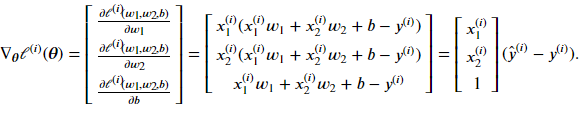### 小结

• 和大多数深度学习模型一样，对于线性回归这样一种单层神经网络，它的基本要素包括模型、训练数据、损失函数和优化算法。
• 既可以用神经网络图表示线性回归，又可以用矢量计算表示该模型。
• 应该尽可能采用矢量计算，以提升计算效率。

### 练习

• 使用其他包（如NumPy）或其他编程语言（如MATLAB），比较相加两个向量的两种方法的运行时间。

## 线性回归的从零开始实现

%matplotlib inline
from IPython import display
from matplotlib import pyplot as plt
import random


### 生成数据集

$\boldsymbol{y} = \boldsymbol{X}\boldsymbol{w} + b + \epsilon,$

num_inputs = 2
num_examples = 1000
true_w = [2, -3.4]
true_b = 4.2
features = nd.random.normal(scale=1, shape=(num_examples, num_inputs))
labels = true_w * features[:, 0] + true_w * features[:, 1] + true_b
labels += nd.random.normal(scale=0.01, shape=labels.shape)


features, labels

(
[2.2122064 0.7740038]
<NDArray 2 @cpu(0)>,
[6.000587]
<NDArray 1 @cpu(0)>)


def use_svg_display():
# 用矢量图显示
display.set_matplotlib_formats('svg')

def set_figsize(figsize=(3.5, 2.5)):
use_svg_display()
# 设置图的尺寸
plt.rcParams['figure.figsize'] = figsize

set_figsize()
plt.scatter(features[:, 1].asnumpy(), labels.asnumpy(), 1);  # 加分号只显示图### 读取数据

# 本函数已保存在d2lzh包中方便以后使用
def data_iter(batch_size, features, labels):
num_examples = len(features)
indices = list(range(num_examples))
random.shuffle(indices)  # 样本的读取顺序是随机的
for i in range(0, num_examples, batch_size):
j = nd.array(indices[i: min(i + batch_size, num_examples)])
yield features.take(j), labels.take(j)  # take函数根据索引返回对应元素


batch_size = 10

for X, y in data_iter(batch_size, features, labels):
print(X, y)
break

[[ 1.8523372  -1.1978964 ]
[-1.464119    0.50711036]
[-0.02477526 -0.3930928 ]
[ 0.5264945  -0.4439822 ]
[-0.65918195 -0.7501681 ]
[ 2.0027666   0.04691195]
[ 0.23753463 -0.16727363]
[-1.2387311   1.6483529 ]
[ 1.3671521   0.4300703 ]
[-0.13764037  3.4004579 ]]
<NDArray 10x2 @cpu(0)>
[11.983968   -0.46103162  5.501128    6.778484    5.426218    8.048336
5.2323737  -3.8805866   5.4759536  -7.6405106 ]
<NDArray 10 @cpu(0)>


### 初始化模型参数

w = nd.random.normal(scale=0.01, shape=(num_inputs, 1))
b = nd.zeros(shape=(1,))


w.attach_grad()


### 定义模型

def linreg(X, w, b):  # 本函数已保存在d2lzh包中方便以后使用
return nd.dot(X, w) + b


### 定义损失函数

def squared_loss(y_hat, y):  # 本函数已保存在d2lzh包中方便以后使用
return (y_hat - y.reshape(y_hat.shape)) ** 2 / 2


### 定义优化算法

def sgd(params, lr, batch_size):  # 本函数已保存在d2lzh包中方便以后使用
for param in params:
param[:] = param - lr * param.grad / batch_size


### 训练模型

lr = 0.03
num_epochs = 3
net = linreg
loss = squared_loss

for epoch in range(num_epochs):  # 训练模型一共需要num_epochs个迭代周期
# 在每一个迭代周期中，会使用训练数据集中所有样本一次（假设样本数能够被批量大小整除）。X
# 和y分别是小批量样本的特征和标签
for X, y in data_iter(batch_size, features, labels):
l = loss(net(X, w, b), y)  # l是有关小批量X和y的损失
l.backward()  # 小批量的损失对模型参数求梯度
sgd([w, b], lr, batch_size)  # 使用小批量随机梯度下降迭代模型参数
train_l = loss(net(features, w, b), labels)
print('epoch %d, loss %f' % (epoch + 1, train_l.mean().asnumpy()))

epoch 1, loss 0.040909
epoch 2, loss 0.000152
epoch 3, loss 0.000050


true_w, w

([2, -3.4],
[[ 1.9996984]
[-3.3998005]]
<NDArray 2x1 @cpu(0)>)

true_b, b

(4.2,
[4.20011]
<NDArray 1 @cpu(0)>)


### 小结

• 可以看出，仅使用NDArrayautograd模块就可以很容易地实现一个模型。接下来，本书会在此基础上描述更多深度学习模型，并介绍怎样使用更简洁的代码（见下一节）来实现它们。

### 练习

• 为什么squared_loss函数中需要使用reshape函数？
• 尝试使用不同的学习率，观察损失函数值的下降快慢。
• 如果样本个数不能被批量大小整除，data_iter函数的行为会有什么变化？

## softmax回归

### 分类问题

#### softmax回归模型

softmax回归跟线性回归一样将输入特征与权重做线性叠加。与线性回归的一个主要不同在于，softmax回归的输出值个数等于标签里的类别数。因为一共有4种特征和3种输出动物类别，所以权重包含12个标量（带下标的$$w$$）、偏差包含3个标量（带下标的$$b$$），且对每个输入计算$$o_1, o_2, o_3$$这3个输出：

\begin{aligned} o_1 &= x_1 w_{11} + x_2 w_{21} + x_3 w_{31} + x_4 w_{41} + b_1,\\ o_2 &= x_1 w_{12} + x_2 w_{22} + x_3 w_{32} + x_4 w_{42} + b_2,\\ o_3 &= x_1 w_{13} + x_2 w_{23} + x_3 w_{33} + x_4 w_{43} + b_3. \end{aligned}#### softmax运算

softmax运算符（softmax operator）解决了以上两个问题。它通过下式将输出值变换成值为正且和为1的概率分布：

$\hat{y}_1, \hat{y}_2, \hat{y}_3 = \text{softmax}(o_1, o_2, o_3),$

$\hat{y}_1 = \frac{ \exp(o_1)}{\sum_{i=1}^3 \exp(o_i)},\quad \hat{y}_2 = \frac{ \exp(o_2)}{\sum_{i=1}^3 \exp(o_i)},\quad \hat{y}_3 = \frac{ \exp(o_3)}{\sum_{i=1}^3 \exp(o_i)}.$

$\operatorname*{argmax}_i o_i = \operatorname*{argmax}_i \hat y_i,$

### 单样本分类的矢量计算表达式

$\boldsymbol{W} = \begin{bmatrix} w_{11} & w_{12} & w_{13} \\ w_{21} & w_{22} & w_{23} \\ w_{31} & w_{32} & w_{33} \\ w_{41} & w_{42} & w_{43} \end{bmatrix},\quad \boldsymbol{b} = \begin{bmatrix} b_1 & b_2 & b_3 \end{bmatrix},$

$\boldsymbol{x}^{(i)} = \begin{bmatrix}x_1^{(i)} & x_2^{(i)} & x_3^{(i)} & x_4^{(i)}\end{bmatrix},$

$\boldsymbol{o}^{(i)} = \begin{bmatrix}o_1^{(i)} & o_2^{(i)} & o_3^{(i)}\end{bmatrix},$

$\boldsymbol{\hat{y}}^{(i)} = \begin{bmatrix}\hat{y}_1^{(i)} & \hat{y}_2^{(i)} & \hat{y}_3^{(i)}\end{bmatrix}.$

softmax回归对样本$$i$$分类的矢量计算表达式为

\begin{aligned} \boldsymbol{o}^{(i)} &= \boldsymbol{x}^{(i)} \boldsymbol{W} + \boldsymbol{b},\\ \boldsymbol{\hat{y}}^{(i)} &= \text{softmax}(\boldsymbol{o}^{(i)}). \end{aligned}

### 小批量样本分类的矢量计算表达式

\begin{aligned} \boldsymbol{O} &= \boldsymbol{X} \boldsymbol{W} + \boldsymbol{b},\\ \boldsymbol{\hat{Y}} &= \text{softmax}(\boldsymbol{O}), \end{aligned}

### 交叉熵损失函数

$H\left(\boldsymbol y^{(i)}, \boldsymbol {\hat y}^{(i)}\right ) = -\sum_{j=1}^q y_j^{(i)} \log \hat y_j^{(i)},$

$\ell(\boldsymbol{\Theta}) = \frac{1}{n} \sum_{i=1}^n H\left(\boldsymbol y^{(i)}, \boldsymbol {\hat y}^{(i)}\right ),$

### 小结

• softmax回归适用于分类问题。它使用softmax运算输出类别的概率分布。
• softmax回归是一个单层神经网络，输出个数等于分类问题中的类别个数。
• 交叉熵适合衡量两个概率分布的差异。

### 练习

• 查阅资料，了解最大似然估计。它与最小化交叉熵损失函数有哪些异曲同工之妙？

## softmax回归的从零开始实现

%matplotlib inline
import d2lzh as d2l


### 获取和读取数据

batch_size = 256


### 初始化模型参数

num_inputs = 784
num_outputs = 10

W = nd.random.normal(scale=0.01, shape=(num_inputs, num_outputs))
b = nd.zeros(num_outputs)


W.attach_grad()


### 实现softmax运算

X = nd.array([[1, 2, 3], [4, 5, 6]])
X.sum(axis=0, keepdims=True), X.sum(axis=1, keepdims=True)

(
[[5. 7. 9.]]
<NDArray 1x3 @cpu(0)>,
[[ 6.]
[15.]]
<NDArray 2x1 @cpu(0)>)


def softmax(X):
X_exp = X.exp()
partition = X_exp.sum(axis=1, keepdims=True)
return X_exp / partition  # 这里应用了广播机制


X = nd.random.normal(shape=(2, 5))
X_prob = softmax(X)
X_prob, X_prob.sum(axis=1)

(
[[0.21324193 0.33961776 0.1239742  0.27106097 0.05210521]
[0.11462264 0.3461234  0.19401033 0.29583326 0.04941036]]
<NDArray 2x5 @cpu(0)>,
[1.0000001 1.       ]
<NDArray 2 @cpu(0)>)


### 定义模型

def net(X):
return softmax(nd.dot(X.reshape((-1, num_inputs)), W) + b)


### 定义损失函数

y_hat = nd.array([[0.1, 0.3, 0.6], [0.3, 0.2, 0.5]])
y = nd.array([0, 2], dtype='int32')
nd.pick(y_hat, y)

[0.1 0.5]
<NDArray 2 @cpu(0)>


def cross_entropy(y_hat, y):
return -nd.pick(y_hat, y).log()


### 计算分类准确率

def accuracy(y_hat, y):
return (y_hat.argmax(axis=1) == y.astype('float32')).mean().asscalar()


accuracy(y_hat, y)

0.5


# 本函数已保存在d2lzh包中方便以后使用。该函数将被逐步改进：它的完整实现将在“图像增广”一节中
# 描述
def evaluate_accuracy(data_iter, net):
acc_sum, n = 0.0, 0
for X, y in data_iter:
y = y.astype('float32')
acc_sum += (net(X).argmax(axis=1) == y).sum().asscalar()
n += y.size
return acc_sum / n


evaluate_accuracy(test_iter, net)

0.0925


### 训练模型

num_epochs, lr = 5, 0.1

# 本函数已保存在d2lzh包中方便以后使用
def train_ch3(net, train_iter, test_iter, loss, num_epochs, batch_size,
params=None, lr=None, trainer=None):
for epoch in range(num_epochs):
train_l_sum, train_acc_sum, n = 0.0, 0.0, 0
for X, y in train_iter:
y_hat = net(X)
l = loss(y_hat, y).sum()
l.backward()
if trainer is None:
d2l.sgd(params, lr, batch_size)
else:
trainer.step(batch_size)  # “softmax回归的简洁实现”一节将用到
y = y.astype('float32')
train_l_sum += l.asscalar()
train_acc_sum += (y_hat.argmax(axis=1) == y).sum().asscalar()
n += y.size
test_acc = evaluate_accuracy(test_iter, net)
print('epoch %d, loss %.4f, train acc %.3f, test acc %.3f'
% (epoch + 1, train_l_sum / n, train_acc_sum / n, test_acc))

train_ch3(net, train_iter, test_iter, cross_entropy, num_epochs, batch_size,
[W, b], lr)

epoch 1, loss 0.7880, train acc 0.746, test acc 0.794
epoch 2, loss 0.5739, train acc 0.810, test acc 0.827
epoch 3, loss 0.5297, train acc 0.823, test acc 0.832
epoch 4, loss 0.5046, train acc 0.830, test acc 0.838
epoch 5, loss 0.4903, train acc 0.835, test acc 0.838


### 预测

for X, y in test_iter:
break

true_labels = d2l.get_fashion_mnist_labels(y.asnumpy())
pred_labels = d2l.get_fashion_mnist_labels(net(X).argmax(axis=1).asnumpy())
titles = [true + '\n' + pred for true, pred in zip(true_labels, pred_labels)]

d2l.show_fashion_mnist(X[0:9], titles[0:9])### 小结

• 可以使用softmax回归做多类别分类。与训练线性回归相比，你会发现训练softmax回归的步骤和它非常相似：获取并读取数据、定义模型和损失函数并使用优化算法训练模型。事实上，绝大多数深度学习模型的训练都有着类似的步骤。

### 练习

• 在本节中，我们直接按照softmax运算的数学定义来实现softmax函数。这可能会造成什么问题？（提示：试一试计算$$\exp(50)$$的大小。）
• 本节中的cross_entropy函数是按照“softmax回归”一节中的交叉熵损失函数的数学定义实现的。这样的实现方式可能有什么问题？（提示：思考一下对数函数的定义域。）
• 你能想到哪些办法来解决上面的两个问题？

## 模型选择、欠拟合和过拟合

### 欠拟合和过拟合

#### 模型复杂度

$\hat{y} = b + \sum_{k=1}^K x^k w_k$### 多项式函数拟合实验

%matplotlib inline
import d2lzh as d2l
from mxnet import autograd, gluon, nd
from mxnet.gluon import data as gdata, loss as gloss, nn


#### 生成数据集

$y = 1.2x - 3.4x^2 + 5.6x^3 + 5 + \epsilon,$

n_train, n_test, true_w, true_b = 100, 100, [1.2, -3.4, 5.6], 5
features = nd.random.normal(shape=(n_train + n_test, 1))
poly_features = nd.concat(features, nd.power(features, 2),
nd.power(features, 3))
labels = (true_w * poly_features[:, 0] + true_w * poly_features[:, 1]
+ true_w * poly_features[:, 2] + true_b)
labels += nd.random.normal(scale=0.1, shape=labels.shape)


features[:2], poly_features[:2], labels[:2]

(
[[2.2122064]
[0.7740038]]
<NDArray 2x1 @cpu(0)>,
[[ 2.2122064   4.893857   10.826221  ]
[ 0.7740038   0.5990819   0.46369165]]
<NDArray 2x3 @cpu(0)>,
[51.674885   6.3585763]
<NDArray 2 @cpu(0)>)


#### 定义、训练和测试模型

# 本函数已保存在d2lzh包中方便以后使用
def semilogy(x_vals, y_vals, x_label, y_label, x2_vals=None, y2_vals=None,
legend=None, figsize=(3.5, 2.5)):
d2l.set_figsize(figsize)
d2l.plt.xlabel(x_label)
d2l.plt.ylabel(y_label)
d2l.plt.semilogy(x_vals, y_vals)
if x2_vals and y2_vals:
d2l.plt.semilogy(x2_vals, y2_vals, linestyle=':')
d2l.plt.legend(legend)


num_epochs, loss = 100, gloss.L2Loss()

def fit_and_plot(train_features, test_features, train_labels, test_labels):
net = nn.Sequential()
net.initialize()
batch_size = min(10, train_labels.shape)
train_features, train_labels), batch_size, shuffle=True)
trainer = gluon.Trainer(net.collect_params(), 'sgd',
{'learning_rate': 0.01})
train_ls, test_ls = [], []
for _ in range(num_epochs):
for X, y in train_iter:
l = loss(net(X), y)
l.backward()
trainer.step(batch_size)
train_ls.append(loss(net(train_features),
train_labels).mean().asscalar())
test_ls.append(loss(net(test_features),
test_labels).mean().asscalar())
print('final epoch: train loss', train_ls[-1], 'test loss', test_ls[-1])
semilogy(range(1, num_epochs + 1), train_ls, 'epochs', 'loss',
range(1, num_epochs + 1), test_ls, ['train', 'test'])
print('weight:', net.weight.data().asnumpy(),
'\nbias:', net.bias.data().asnumpy())


#### 三阶多项式函数拟合（正常）

fit_and_plot(poly_features[:n_train, :], poly_features[n_train:, :],
labels[:n_train], labels[n_train:])

final epoch: train loss 0.006922996 test loss 0.011304822
weight: [[ 1.3227708 -3.3637862  5.56338  ]]
bias: [4.952137]#### 线性函数拟合（欠拟合）

fit_and_plot(features[:n_train, :], features[n_train:, :], labels[:n_train],
labels[n_train:])

final epoch: train loss 43.99767 test loss 160.7238
weight: [[15.56557]]
bias: [2.282313]#### 训练样本不足（过拟合）

fit_and_plot(poly_features[0:2, :], poly_features[n_train:, :], labels[0:2],
labels[n_train:])

final epoch: train loss 0.4027369 test loss 103.314186
weight: [[1.3872364 1.9376589 3.5085924]]
bias: [1.2312856]### 小结

• 由于无法从训练误差估计泛化误差，一味地降低训练误差并不意味着泛化误差一定会降低。机器学习模型应关注降低泛化误差。
• 可以使用验证数据集来进行模型选择。
• 欠拟合指模型无法得到较低的训练误差，过拟合指模型的训练误差远小于它在测试数据集上的误差。
• 应选择复杂度合适的模型并避免使用过少的训练样本。

### 练习

• 如果用一个三阶多项式模型来拟合一个线性模型生成的数据，可能会有什么问题？为什么？
• 在本节提到的三阶多项式拟合问题里，有没有可能把100个样本的训练误差的期望降到0，为什么？（提示：考虑噪声项的存在。）
]]>
<![CDATA[深夜，来谈一谈未来]]> 2019-10-05T23:30:31+08:00 https://zning.me/15702894319169.html 国庆已经过半，近期对于未来的科技发展，有些许想法从头脑中迸出。趁着假期这个机会分享给大家。

## 神经网络与深度学习的终结：始于硬件 败于“软件”## 弱化测试运维的开发的命运走向何方？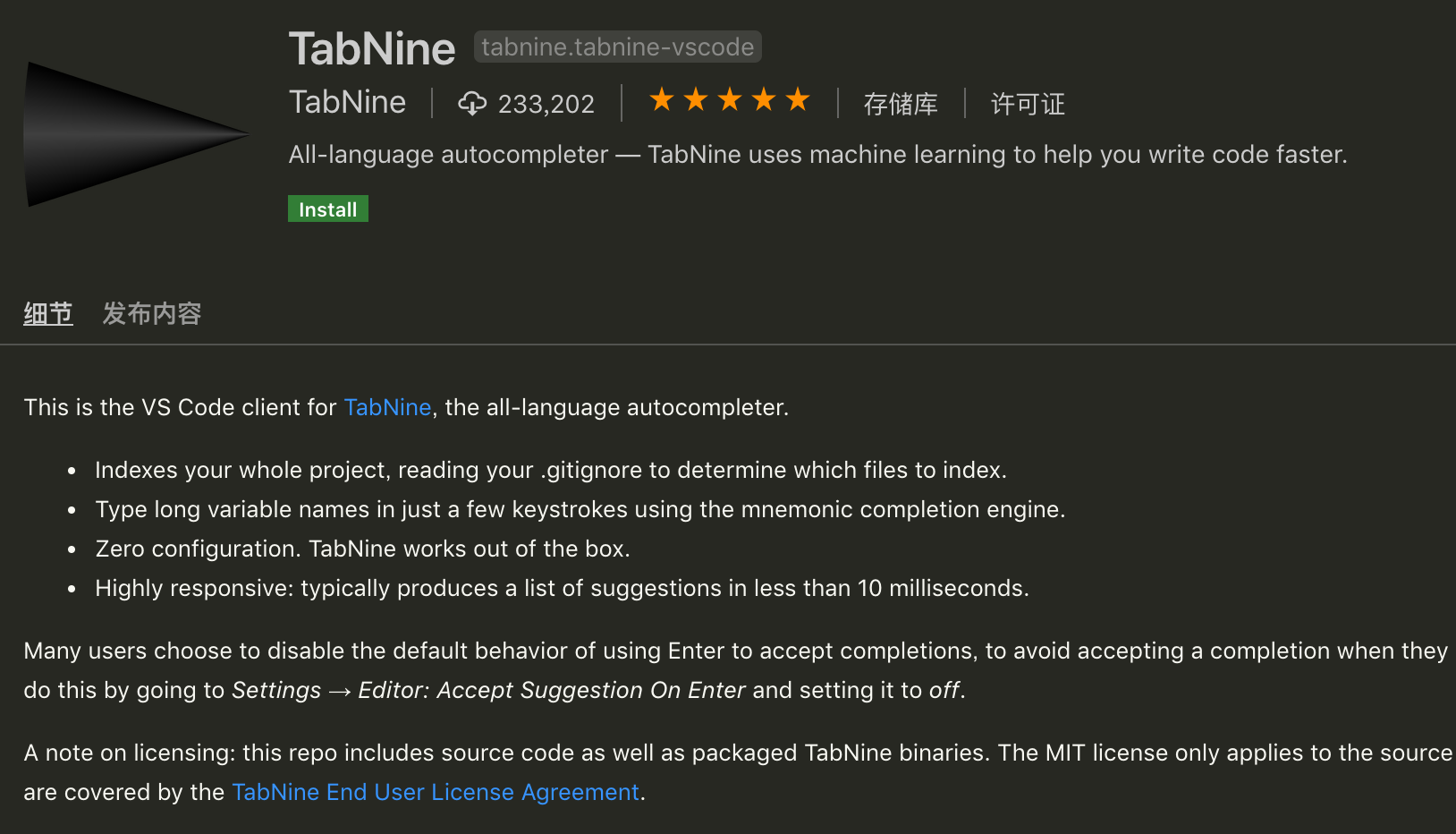VS Code插件TabNine介绍截图

## 算法工程师: 高精尖的岗位 稳就业的救星]]>
<![CDATA[深度学习之《深度学习入门》学习笔记（四）神经网络的学习]]> 2019-09-22T10:52:11+08:00 https://zning.me/15691207315042.html 最近学习吴恩达《Machine Learning》课程以及《深度学习入门：基于Python的理论与实现》书，一些东西总结了下。现就后者学习进行笔记总结。本文是本书的学习笔记（四）神经网络的学习。

## 损失函数

### 均方误差

$E = \frac{1}{2} \sum_{k}^{}(y_{k}-t_{k})^2$

$$y_{k}$$表示神经网络的输出，$$t_{k}$$表示监督数据，$$k$$表示数据的维度。其中，$$t$$表示监督数据，将正确的解标签设为1，其他均设为0，其他标签表示为0的表示方法称为one-hot表示

# 均方误差定义函数
def mean_squared_error(y, t):
return 0.5 * np.sum((y-t)**2)

# 设“2”为正解
t = [0, 0, 1, 0, 0, 0, 0, 0, 0, 0]

#例1：“2”的概率最高的情况（0.6）
y = [0.1, 0.05, 0.6, 0.0, 0.05, 0.1, 0.0, 0.1, 0.0, 0.0]
mean_squared_error(np.array(y),np.array(t))


0.09750000000000003

# 例2：“7”的概率最高的情况（0.6）
y = [0.1, 0.05, 0.1, 0.0, 0.05, 0.1, 0.0, 0.6, 0.0, 0.0]

mean_squared_error(np.array(y),np.array(t))


0.5975

### 交叉熵误差

$E=-\sum_{k}^{} t_{k} ln y_{k}$

$$y_{k}$$是神经网络的输出，$$t_{k}$$是正确解标签。并且，中只有正确解标签的索引为1，其他均为0（one-hot表示）。交叉熵误差的值是由正确解标签所对应的输出结果决定的。

# 实现交叉熵误差
def cross_entropy_error0(y, t):
delta = 1e-7
return -np.sum(t * np.log(y + delta))


# 进行简单计算
# 设“2”为正解
t = [0, 0, 1, 0, 0, 0, 0, 0, 0, 0]
#例1：“2”的概率最高的情况（0.6）
y = [0.1, 0.05, 0.6, 0.0, 0.05, 0.1, 0.0, 0.1, 0.0, 0.0]
cross_entropy_error0(np.array(y),np.array(t))


0.510825457099338

# 例2：“7”的概率最高的情况（0.6）
y = [0.1, 0.05, 0.1, 0.0, 0.05, 0.1, 0.0, 0.6, 0.0, 0.0]
cross_entropy_error0(np.array(y),np.array(t))


2.302584092994546

### mini-batch学习

$E=-\frac{1}{N} \sum_{n}^{} \sum_{k}^{} t_{nk} ln y_{nk}$

# 读入MNIST 代码暂略
import sys, os
sys.path.append(os.pardir)
import numpy as np

# 使用np.random.choice进行随机选取
np.random.choice(60000, 10)


array([ 5332, 11993, 16553, 47954, 31537, 4750, 52005, 1159, 6775,
46043])

# 可同时处理单个和批量数据
def cross_entropy_error1(y, t):
if y.nidm == 1:
t = t.reshape(1, t.size)
y = y.reshape(1, y.size)

delta = 1e-7
batch_size = y.shape
return -np.sum(t * np.log(y + delta)) / batch_size


# 可同时处理单个和批量数据
def cross_entropy_error2(y, t):
if y.nidm == 1:
t = t.reshape(1, t.size)
y = y.reshape(1, y.size)

delta = 1e-7
batch_size = y.shape
return -np.sum(np.log(y[np.arange(batch_size), t] + delta)) / batch_size


### 为什么要设定损失函数

Q: 为什么要导入损失函数？既然我们的目标是获得识别精度尽可能高的神经网络，那不是应该把识别精度作为指标吗？

A: 在神经网络的学习中，寻找最优参数（权重和偏置）时，要寻找使损失函数的值尽可能小的参数。为了找到使损失函数的值尽可能小的地方，需要计算的参数的导数（确切的讲是梯度），然后以这个导数为指引，逐步更新参数的值。而对权重参数的损失函数求导，表示的是“如果稍微改变这个权重参数的值，损失函数的值会如何变化”。如果导数的值为负如，通过使该权重参数向正方向改变，可以减小损失函数的值；反过来，如果导数的值为正如，则通过使该权重参数向负方向改变，可以减小损失函数的值。当导数的值为0时如，无论权重参数向哪个方向变化，损失函数的值都不会改变，此时该权重参数的更新会停在此处。

## 数值微分

### 导数

Python中的舍入误差：

np.float32(1e-50)


0.0

# 对舍入误差减小与使用中心差分实现函数导数程序
def numerical_diff(f, x):
h = 1e-4 #0.0001
return (f(x+h) - f(x-h)) / (2*h)


### 数值微分的例子

# 实现例子
def function_1(x):
return 0.01*x**2 + 0.1*x

import numpy as np
import matplotlib.pylab as plt

x = np.arange(0.0, 20.0, 0.1) #以0.1为单位，从0到20的数组x
y = function_1(x)
plt.xlabel("x")
plt.ylabel("y")
plt.plot(x, y)
plt.show()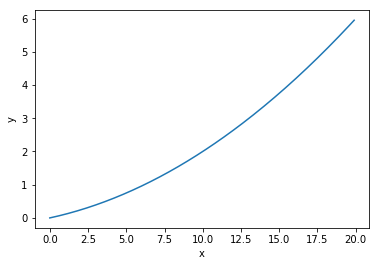#计算上面式子的5,10处导数
numerical_diff(function_1, 5)


0.1999999999990898

numerical_diff(function_1, 10)


0.2999999999986347

def tangent_line(f, x):
d = numerical_diff(f, x)
print(d)
y = f(x) - d*x
return lambda t: d*t + y

x = np.arange(0.0, 20.0, 0.1)
y = function_1(x)
plt.xlabel("x")
plt.ylabel("f(x)")

tf = tangent_line(function_1, 5)
tf2 = tangent_line(function_1, 10)
y2 = tf(x)
y22 = tf2(x)

plt.plot(x, y)
plt.plot(x, y2)
plt.show()
plt.plot(x, y)
plt.plot(x, y22)
plt.show()


0.1999999999990898
0.2999999999986347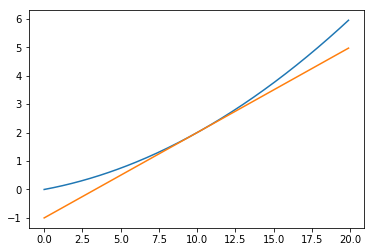### 偏导数

# 实现上式的代码
def function_2(x):
#或者return np.sum(x**2)
return x**2 + x**2

# 求偏导1
def function_tmp1(x0):
return x0*x0 + 4.0**2.0

numerical_diff(function_tmp1, 3.0)


6.00000000000378

# 求偏导2
def function_tmp2(x1):
return 3.0**2.0 + x1*x1

numerical_diff(function_tmp1, 4.0)


7.999999999999119

## 梯度

# 梯度的代码实现
h = 1e-4 #0.001

for idx in range(x.size):
tmp_val = x[idx]
# f(x+h)的计算
x[idx] = tmp_val + h
fxh1 = f(x)

# f(x-h)的计算
x[idx] = tmp_val - h
fxh2 = f(x)

grad[idx] = (fxh1 - fxh2) / (2*h)
x[idx] = tmp_val # 还原值



# 求点(3,4) (0,2) (3,0)处的梯度


array([6., 8.])

numerical_gradient(function_2, np.array([0.0, 2.0]))


array([0., 4.])

numerical_gradient(function_2, np.array([3.0, 0.0]))


array([6., 0.])

import numpy as np
import matplotlib.pylab as plt
from mpl_toolkits.mplot3d import Axes3D

h = 1e-4 # 0.0001

for idx in range(x.size):
tmp_val = x[idx]
x[idx] = float(tmp_val) + h
fxh1 = f(x) # f(x+h)

x[idx] = tmp_val - h
fxh2 = f(x) # f(x-h)
grad[idx] = (fxh1 - fxh2) / (2*h)

x[idx] = tmp_val # 还原值

if X.ndim == 1:
else:

for idx, x in enumerate(X):

def function_2(x):
if x.ndim == 1:
return np.sum(x**2)
else:
return np.sum(x**2, axis=1)

def tangent_line(f, x):
print(d)
y = f(x) - d*x
return lambda t: d*t + y

if __name__ == '__main__':
x0 = np.arange(-2, 2.5, 0.25)
x1 = np.arange(-2, 2.5, 0.25)
X, Y = np.meshgrid(x0, x1)

X = X.flatten()
Y = Y.flatten()

plt.figure()
plt.xlim([-2, 2])
plt.ylim([-2, 2])
plt.xlabel('x0')
plt.ylabel('x1')
plt.grid()
plt.legend()
plt.draw()
plt.show()### 梯度法

$x_{0}=x_{0}-\eta \frac{\partial f}{\partial x_{0}}$

$x_{1}=x_{1}-\eta \frac{\partial f}{\partial x_{1}}$

def gradient_descent(f, init_x, lr=0.01, step_num=100):
"""
参数f是要进行最优化的函数，init_x是初始值，lr是学习率learning rate，step_num是梯度法的重复次数。
"""
x = init_x
x_history = []

for i in range(step_num):
x_history.append( x.copy() )

return x, np.array(x_history)

# 用梯度法求f(x0+x1)=x0^2+x1^2的最小值
def function_2(x):
return x**2 + x**2

init_x = np.array([-3.0, 4.0])
x, x_history = gradient_descent(function_2, init_x=init_x, lr=0.1, step_num=100)

# 用图像表示上面的函数梯度下降法的步骤
plt.plot( [-5, 5], [0,0], '--b')
plt.plot( [0,0], [-5, 5], '--b')
plt.plot(x_history[:,0], x_history[:,1], 'o')

plt.xlim(-3.5, 3.5)
plt.ylim(-4.5, 4.5)
plt.xlabel("X0")
plt.ylabel("X1")
plt.show()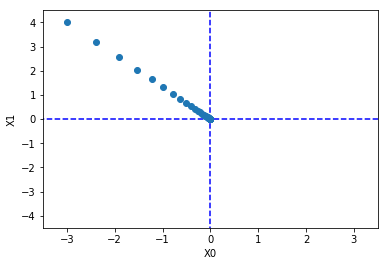# 学习率过大的例子：lr=10.0
init_x = np.array([-3.0, 4.0])
x, x_history = gradient_descent(function_2, init_x=init_x, lr=10.0, step_num=100)
x


array([-2.58983747e+13, -1.29524862e+12])

# 学习率过小的例子：lr=1e-10
init_x = np.array([-3.0, 4.0])
x, x_history = gradient_descent(function_2, init_x=init_x, lr=1e-10, step_num=100)
x


array([-2.99999994, 3.99999992])

### 神经网络的梯度

# 一个简单的神经网络
# coding: utf-8
import sys, os
sys.path.append('../input/deeplearningfromscratch/deeplearningfromscratch')  # 为了导入父目录中的文件而进行的设定
import numpy as np
from common.functions import softmax, cross_entropy_error

class simpleNet:
def __init__(self):
self.W = np.random.randn(2,3)

def predict(self, x):
return np.dot(x, self.W)

def loss(self, x, t):
z = self.predict(x)
y = softmax(z)
loss = cross_entropy_error(y, t)

return loss

net = simpleNet()
print(net.W)

x = np.array([0.6, 0.9])
p = net.predict(x)
print(p)

print(np.argmax(p))

t = np.array([0, 0, 1]) # 正确解标签

f = lambda w: net.loss(x, t)

print(dW)


[[ 0.61060921 0.39278321 -0.19230499]
[-0.57160886 0.59945886 -0.26529107]]
[-0.14808245 0.7751829 -0.35414496]
1
[[ 0.13852712 0.34874166 -0.48726878]
[ 0.20779067 0.5231125 -0.73090317]]

## 学习算法的实现

1. mini-batch：从训练数据中随机选出一部分数据，这部分数据称为mini-batch。我们的目标是减小mini-batch的损失函数的值。
2. 计算梯度：为了减小mini-batch的损失函数的值，需要求出各个权重参数的梯度。梯度表示损失函数的值减小最多的方向。
3. 更新参数：将权重参数沿梯度方向进行微小更新。
4. 重复：重复第1、2、3步。

### 2层神经网络的类

from common.functions import *

class TwoLayerNet:

def __init__(self, input_size, hidden_size, output_size, weight_init_std=0.01):
# 初始化权重
self.params = {}
self.params['W1'] = weight_init_std * np.random.randn(input_size, hidden_size)
self.params['b1'] = np.zeros(hidden_size)
self.params['W2'] = weight_init_std * np.random.randn(hidden_size, output_size)
self.params['b2'] = np.zeros(output_size)

def predict(self, x):
W1, W2 = self.params['W1'], self.params['W2']
b1, b2 = self.params['b1'], self.params['b2']

a1 = np.dot(x, W1) + b1
z1 = sigmoid(a1)
a2 = np.dot(z1, W2) + b2
y = softmax(a2)

return y

# x:输入数据, t:监督数据
def loss(self, x, t):
y = self.predict(x)

return cross_entropy_error(y, t)

def accuracy(self, x, t):
y = self.predict(x)
y = np.argmax(y, axis=1)
t = np.argmax(t, axis=1)

accuracy = np.sum(y == t) / float(x.shape)
return accuracy

# x:输入数据, t:监督数据
loss_W = lambda W: self.loss(x, t)

W1, W2 = self.params['W1'], self.params['W2']
b1, b2 = self.params['b1'], self.params['b2']

batch_num = x.shape

# forward
a1 = np.dot(x, W1) + b1
z1 = sigmoid(a1)
a2 = np.dot(z1, W2) + b2
y = softmax(a2)

# backward
dy = (y - t) / batch_num

da1 = np.dot(dy, W2.T)


# 二层神经网络例子
net = TwoLayerNet(input_size = 784, hidden_size = 100, output_size = 10)
print(net.params['W1'].shape)
print(net.params['b1'].shape)
print(net.params['W2'].shape)
print(net.params['b2'].shape)


(784, 100)
(100,)
(100, 10)
(10,)

#推理处理的实现如下
x = np.random.rand(100, 784) # 伪输入数据100笔
y = net.predict(x)
t = np.random.rand(100, 10) # 伪正确解标签10笔



(784, 100)
(100,)
(100, 10)
(10,)

1. __init__(self, input_size, hidden_size, output_size)：类的初始化方法，参数依次表示输入层的神经元数、隐藏层的神经元数、输出层的神经元数。

因为进行手写数字识别时，输入图像的大小是784(28x28)，输出为10个类别，所以指定参数input_size = 784, output_size = 10，将隐藏层的个数, hidden_size设置为一个合适的值即可（例如这里是100）。

2. predict(self, x)、accuracy(self, x, t)同上一章神经网络的推理处理基本一致。

3. loss(self, x, t)是计算损失函数值的方法。这个方法会基于predict()的结果和正确解标签，计算交叉熵误差。

4. numerical_gradient(self, x, t)是计算各个参数的梯度，而gradient(self, x, t)是使用误差反向传播法高效计算梯度的方法。

numerical_gradient(self, x, t)是基于数值微分计算参数的梯度。而gradient(self, x, t)是使用误差反向传播法高速计算梯度，其求到的梯度和数值微分的结果基本一致，且速度比前者快。

### mini-batch的实现

import numpy as np
import matplotlib.pyplot as plt
# from two_layer_net import TwoLayerNet

# 读入数据
(x_train, t_train), (x_test, t_test) = load_mnist(normalize=True, one_hot_label=True)

network = TwoLayerNet(input_size=784, hidden_size=50, output_size=10)

# 超参数
iters_num = 10000  # 适当设定循环的次数
train_size = x_train.shape
batch_size = 100
learning_rate = 0.1

train_loss_list = []
train_acc_list = []
test_acc_list = []

# 平均每个epoch的重复次数
iter_per_epoch = max(train_size / batch_size, 1)

for i in range(iters_num):
# 获取mini-batch

# 计算梯度

# 更新参数
for key in ('W1', 'b1', 'W2', 'b2'):

# 记录学习过程
loss = network.loss(x_batch, t_batch)
train_loss_list.append(loss)

# 计算每个epoch的识别精度
if i % iter_per_epoch == 0:
train_acc = network.accuracy(x_train, t_train)
test_acc = network.accuracy(x_test, t_test)
train_acc_list.append(train_acc)
test_acc_list.append(test_acc)
print("train acc, test acc | " + str(train_acc) + ", " + str(test_acc))

# 绘制图形
markers = {'train': 'o', 'test': 's'}
x = np.arange(len(train_acc_list))
plt.plot(x, train_acc_list, label='train acc')
plt.plot(x, test_acc_list, label='test acc', linestyle='--')
plt.xlabel("epochs")
plt.ylabel("accuracy")
plt.ylim(0, 1.0)
plt.legend(loc='lower right')
plt.show()


train acc, test acc | 0.10441666666666667, 0.1028
train acc, test acc | 0.7994333333333333, 0.8037
train acc, test acc | 0.8809833333333333, 0.8833
train acc, test acc | 0.9003666666666666, 0.9024
train acc, test acc | 0.9098833333333334, 0.9125
train acc, test acc | 0.9164, 0.9178
train acc, test acc | 0.9214, 0.9244
train acc, test acc | 0.92525, 0.9277
train acc, test acc | 0.92875, 0.9321
train acc, test acc | 0.9321166666666667, 0.9337
train acc, test acc | 0.9348333333333333, 0.9358
train acc, test acc | 0.93755, 0.9384
train acc, test acc | 0.9400833333333334, 0.9407
train acc, test acc | 0.9424166666666667, 0.9422
train acc, test acc | 0.9445666666666667, 0.9431
train acc, test acc | 0.9466666666666667, 0.9459
train acc, test acc | 0.9480833333333333, 0.9479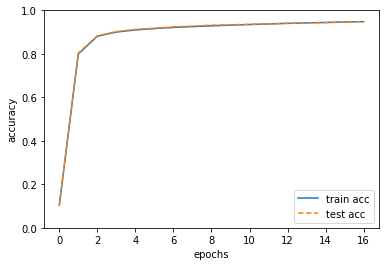epoch是一个单位，一个epoch表示学习中所有训练数据均被使用过一次时的更新次数。

]]>
<![CDATA[架构学习书摘总结（五）架构实战]]> 2019-06-08T23:24:16+08:00 https://zning.me/15600074562241.html 最近阅读了一本架构方面的入门图书叫《从零开始学架构：照着做，你也能成为架构师》，部分内容比较不错，先做书摘总结，以便加深印象与未来回顾学习。

## 第十六章 消息队列设计实战

1. 需求：

1. 用户发微博，通过审核子系统、统计子系统、广告子系统、消息子系统等，系统间通过接口调用，每通知一个新系统，微博子系统就要涉及接口进行测试，效率很低，问题定位很麻烦。还有等级子系统给VIP用户发放奖励、通知客服子系统安排专属服务人员……开发人员不胜其烦。
2. 根因在于架构上各业务子系统强耦合，而消息队列正好可以完成子系统的解耦。经过一分析二讨论三开会四汇报五审批等一系列操作后，消息队列系统立项。
2. 设计流程：

1. 识别复杂度：对架构师来说是一项挑战，如果经验不足，那么只能采取“排查法”，从不同角度逐一进行分析。对于此项目具体分析过程如下：

1. 这个消息队列是否需要高性能：关注每秒数据，即TPS和QPS。再考虑系统的读写并不是完全平均的，设计的目标应该以峰值来计算。峰值一般取平均值的3倍。例如，一天平均每秒写入消息为115条，每秒读取消息数是1150条，则消息对类系统的TPS是345，QPS是3450。另外由于现在基数较低，因此系统的设计目标按照峰值的四倍来计算，即TPS为1380，QPS为13800。TPS为1380并不高，但QPS为13800已经比较高了，因此高性能读取是复杂度之一，但这个高性能要求相比Kafka等系统来说也不是很高。
2. 这个消息队列是否需要高可用：消息队列需要高可用性，包括消息写入、消息存储、消息读取都需要保证高可用性。
3. 这个消息队列是否需要高可扩展性：消息队列的功能很明确，基本无需扩展，因此可扩展性不是这个消息队列的复杂度关键。

综合分析下来，消息队列的复杂性主要体现在几个方面：高性能消息读取、高可用消息写入、高可用消息存储、高可用消息读取。

2. 设计备选方案：采用开源Kafka、集群+MySQL存储、集群+自研存储方案。

3. 评估和选择备选方案

质量属性 引入Kafka MySQL存储 自研存储
性能
复杂度 低，基本开箱可用 中，MySQL存储和复制，方案只需要开发服务器集群就可以 高，自研存储方案复杂度很高
硬件成本 高，一个分区就4台机器 低，和Kafka一样
可运维性 低，无法融入现有的运维体系，且运维团队无Scala经验 高，可以融入现有运维体系，MySQL运维很成熟 高，可以融入现有运维体系，并且只需要维护服务器即可，无需维护MySQL
可靠性 高，程序开源方案 高，MySQL存储很成熟 低，自研存储系统可靠性在最初阶段难以保证
人力投入 低，开箱即用 中，只需要开发服务器集群 高，需要开发服务器集群和存储系统

最终根据讨论与架构师决策，选择了备选方案2。

4. 细化方案：在备选方案的基础上进一步细化。

## 第十七章 互联网架构演进

1. 什么样的策略才是真正有效的技术发展策略这个问题让人困惑关键的原因在于不管是潮流派（紧跟技术潮流）、保守派（新技术抱有戒备心，稳定压倒一切），还是跟分派（跟着竞争对手的步子走），都是站在技术本身的角度来考虑问题的，正所谓“不识庐山真面目，只缘身在此山中”。因此，要想看到“庐山真面目”，只有跳出技术的范畴，从一个更广更高的角度——企业的业务发展来考虑这个问题。
2. 企业的发展，归根结底就是业务的发展，而影响一个企业的发展主要有3个因素：市场、技术、管理。在这个铁三角当中，业务处于三角形的中心，毫不夸张的说，市场、技术、管理都是为了支撑企业业务的发展。
3. 我们可以简单地将企业的业务分为两类：产品类和服务类。
4. 对于产品类服务，技术创新推动业务发展。对于服务类服务，业务发展推动技术发展。两类不同关系的原因在于用户选择服务的根本驱动力与选择产品的不同。用户选择一个产品的根本驱动力是其“功能”，而用户选择一个服务的根本驱动力却为“规模”。
5. 当“规模”成为业务的决定因素后，服务模式的创新就成为业务发展的核心驱动力，而产品只是为了完成服务而提供给用户使用的一个载体。
6. 服务类的业务发展路径：提出一种创新的服务模式→吸引了一批用户→业务开始发展→吸引了更多用户→服务模式不断完善和创新→吸引越来越多的用户。
7. 即使是产品类业务，在技术创新开创了一个新的业务后，后续的业务发展也会反向推动技术的发展。
8. 对于架构师来说，判断业务当前和接下来一段时间的主要复杂度是什么是非常关键的。架构师具体应该按照基于业务发展阶段的标准进行判断，这也是为什么架构师必须具备业务理解能力的原因。
9. 互联网业务具有“规模决定一切”的相同点。
10. 互联网业务发展一般分为几个时期：初创期、快速发展期、竞争期、成熟期。不同时期的差别主要体现在两个方面：复杂性、用户规模。
11. 互联网发展的第一个主要方向是“业务越来越复杂”。

初创期业务重点在于创新，只有随着越来越多的用户的使用，通过快速迭代试错、用户的反馈等手段，不断地在实践中去完善，去继续创新。

发展期主要是将原来不完善的业务逐渐完善，因此会有越来越多的新功能不断地加入系统中。对于绝大部分技术团队来说，这个阶段技术的核心工作是快速地实现各种需求，只有这样才能满足业务发展的需要。

架构期进行架构调整，架构期可以用的手段很多，但归根结底可以总结为一个字“拆”，功能、数据库、服务器等什么地方都可以拆。

竞争期对技术的要求更上一层楼的“快”了，新业务的创新给技术带来的典型压力就是新的系统会更多，同时，原有的系统也会拆得越来越多。两者合力的一个典型后果就是系统数量在原来的基础上又增加了很多。而系统越来越多到了一个临界点后就产生到了质变，即系统数量的量变带来了技术工作的质变。主要有重复造轮子、系统交互一团麻。这个时期技术工作的主要解决手段有平台化（存储平台化、数据库平台化、缓存平台化）、服务化（消息队列、服务框架等）。

成熟期进行逐项优化。

12. 互联网发展的第二个主要方向是“用户量越来越”大。主要影响体现在：性能要求越来越高、可用性要求越来越高。

性能：再简单的查询，再高的硬件配置，单台MySQL机器支撑的TPS和QPS最高也就是万级，低的可能是几千，高的也不过几万。分布式将会带来复杂度的大幅度上升。以MySQL为例，分布式MySQL要考虑分库分表、读写分离、复制、同步等很多问题。

可用性：除了口碑，可用性对收入的影响也会随着用户量增大而增大。1万用户宕机1小时，可能才损失了几千元，100万用户宕机10分钟，损失可能就是几十万元了。

## 第十八章 互联网架构模板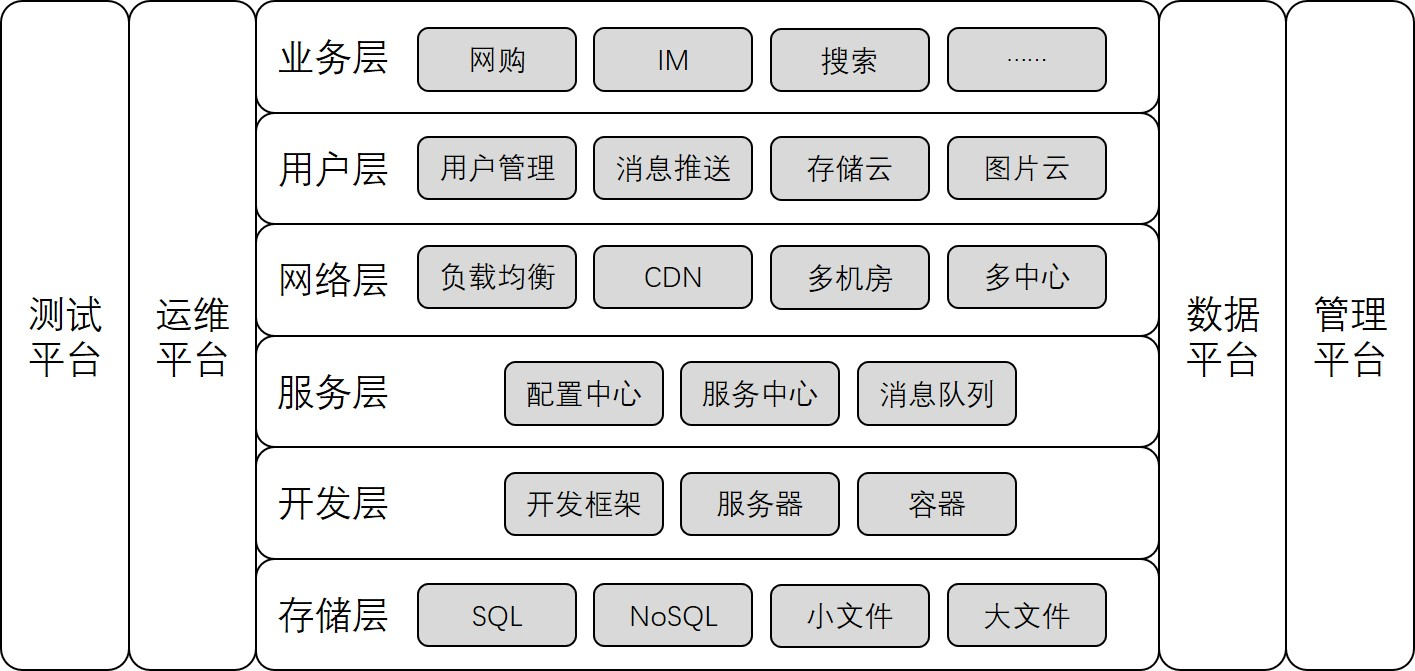1. 存储层技术

1. SQL

SQL即我们通常所说的关系型数据(库)。随着互联网业务的发展，性能要求越来越高，必然面对一个问题：将数据拆分到多个数据库实例才能满足业务的性能需求（其实Oracle也一样，只是时间早晚的问题）。数据库拆分满足了性能的要求，但带来了复杂度的问题：数据如何拆分、数据如何组合。所以互联网公司流行的做法是业务发展到一定阶段后，就会将这部分功能独立成中间件，例如淘宝的TDDL。再后期会导致新的复杂度问题：数据库资源使用率不高，比较浪费以及各SQL集群分开维护，投入的维护成本越来越高。因此，实力雄厚的大公司此时一般都会在SQL集群上构建SQL存储平台，以对业务透明的形式提供资源分配、数据备份、迁移、容灾、读写分离、分库分表等一系列服务。例如，淘宝的UMP(Unified MySQL Platform)系统。

2. NoSQL

NoSQL不是No SQL，而是Not Only SQL，即NoSQL是SQL的补充。首先NoSQL在数据结构上与传统的SQL的不同，例如典型的Memcache的key-value结构、Redis的复杂数据结构、MongoDB的文档数据结构；其次，NoSQL无一例外地都会将性能作为自己的一大卖点。

NoSQL的这两个特点很好地弥补了关系数据库的不足，因此在互联网行业NoSQL的应用基本上是基础要求。

由于NoSQL方案一般自己本身就提供集群功能，因此NoSQL在刚才是应用时很方便，不像SQL分库分表那么复杂。而NoSQL发展到一定规模后，通常都会在NoSQL集群的基础之上再实现统一存储平台。同意存储平台主要实现资源动态按需动态分配、资源自动化管理、故障自动化处理等几个功能。当然要发展到这个阶段，一般也是大公司才会这么做，简单来说就是如果只有几十台NoSQL服务器，那么做存储平台的收益不大；但如果有几千台NoSQL服务器，那么NoSQL存储平台就能够产生很大的收益。

3. 小文件存储

4. 大文件存储

2. 开发层技术

1. 开发框架

互联网公司都会指定一个大的技术方向，然后使用统一的开发框架。例如，Java相关的开发框架SSH、SpringMVC、SpringCloud、Play，Ruby的Ruby on Rails，PHP的ThinkPHP，Python的Django等等。使用统一的开发框架能够解决上面提到的各种问题，大大提升组织和团队的开发效率。

对于框架的选择，有一个总的原则：优选成熟的框架，避免盲目追逐新技术。首先，成熟的框架资料文档齐备；其次，成熟的框架受众更广，招聘时更容易招到合适的人才；最后，成熟的框架更加稳定，不会出现大的变动，适合长期发展。

2. Web服务器

开发框架只是负责完成业务功能的开发，真正能够运行起来，给用户提供服务，还需要服务器配合。选择一个服务器主要和开发语言相关，例如Java有Tomcat、JBoss、Resin等，PHP/Python的用Nginx，当然最保险的就是用Apache了，什么语言都支持。有人要担心Apache性能之类的问题，其实不用过早担心这个，等到业务真的发展到Apache撑不住的时候再考虑切换也不迟，那时候你有的是钱，有的是人，有的是时间。

3. 容器

传统的虚拟化技术是虚拟机，解决了跨平台的问题，但由于虚拟机太庞大，启动慢，运行时太占资源，在互联网行业并没有大规模地应用；而Docker的容器技术，虽然没有跨平台，但启动快，几乎不占资源，推出后立刻就火起来了。不要以为Docker只是一个虚拟化或容器技术，它将在很大程度上改变目前的技术形势：

1. 运维方式会发生革命性的变化：Docker启动快，几乎不占资源，随时启动停止，基于Docker打造自动化运维、智能化运维将成为主流方式。
2. 设计模式会发生本质上的变化：启动一个新的容器实例代价如此低，将鼓励设计思路朝“微服务”的方向发展。

例如，一个传统的网站包括登录注册、页面访问、搜索等功能，没有用容器的情况下，除非有特别大的访问量，否则这些功能开始时都是集成在一个系统里面的；有了容器技术以后，一开始就可以将这些功能按照服务的方式设计，避免后续访问量增大时又要重构系统。

3. 服务层技术

服务层的主要目标是为了降低系统间相互关联的复杂度。

1. 配置中心

配置中心就是集中管理各个系统的配置。将配置中心做成通用的系统有如下好处：

1. 集中配置多个系统，操作效率高。
2. 所有配置都在一个集中的地方，检查方便，协作效率高。
3. 配置中心可以实现程序化的规则检查，避免常见的错误。
4. 配置中心相当于备份了系统的配置，当某些情况下需要搭建新的环境时，能够快速搭建环境和恢复业务。

配置中心通过“系统标示+host+port”来标示唯一一个系统运行实例是常见的设计方法。

2. 服务中心

服务中心未来解决跨系统依赖的配置和调度问题。其实现一般来说有两种方式：服务名字系统和服务总线系统。

服务名字系统（Service Name System），类似于DNS，其是为了将Service名称解析为“host+port+接口名称”，但是和DNS一样，真正发起请求的还是请求方。

服务总线系统（Service Bus System），类似于计算机总线，其为了直接又总线系统完成调用，服务请求方不需要直接和服务提供方交互。

两者简单对比如下：

服务总线系统 服务名字系统
复杂度 设计更加复杂，要同时完成配置和调度功能，且本身高性能和高可用的设计也更加复杂。 设计简单，基本类似一个服务配置中心，如果要做调度，需要独立的SDK包。
可用性 可用性的关键，它故障后所有业务间的访问都鼓掌，影响较大，但因为服务总线主要做调度，可以部署两套或多套并行系统。 仅仅保存配置，屌用还是由服务请求方发起，可用性要求没那么高，即使故障，各系统也可以使用本地缓存配置继续完成调用。
灵活性 控制所有的调度和配置，可以做得非常灵活。 仅仅有配置，即使提供独立的SDK支持调度，灵活性也要差一点，毕竟SDK只能获取静态的配置信息。
实时性 系统完成实际的调度，可以做到非常实时，例如某个服务及机器故障后立刻剔除故障节点。 提供调度的SDK包，也需要定时更新配置，不能每次请求都去获取一下最新的配置，实时性一般，这个问题和DNS类似。
可维护性 服务总线系统的修改和升级只需要自己完成即可。 修改和升级大部分情况下要修改SDK包（例如，调度算法变更），修改SDK包要求所有系统应用新SDK包才能生效。
多语言支持 服务总线系统支持通用的HTTP和TCP协议，和语言无关。 服务名字系统提供的SDK包需要适配多个语言，这个工作量也不小。
3. 消息队列

消息队列系统基本功能的实现比较简单，但要做到高性能、高可用、消息时序性、消息事务性则比较难。

4. 网络层技术

1. 负载均衡

负载均衡就是将请求均衡的分配到多个系统上。其实现方式很多，可大可小，可以软件实现，也可以硬件实现。

DNS是最简单也是最常见的负载均衡方式，一般用来实现地理级别的均衡。DNS负载均衡的有点事通用、成本低，但缺点也明显：DNS缓存时间比较长；DNS不能感知后端服务器的状态，只能根据配置策略进行负载均衡，无法做到更加灵活的负载均衡策略。所以对于时延和故障敏感的业务，有一些公司自己实现了HTTP-DNS的功能，即使用HTTP协议实现一个私有的DNS系统，这样的方案与通用DNS优缺点正好相反。

Nginx&LVS&F5用于同一地点内及其级别的负载均衡，其中Nginx是软件的7层负载均衡，LVS是内核的4层负载均衡，F5是硬件做4层负载均衡。

软件和硬件的区别就在于性能，硬件远远高于软件，Nginx的性能是万级，一般的Linux服务器上装个Nginx大概能到5万/秒；LVS的性能是十万级，没有具体测试过，据说可以达到80万/秒；F5性能是百万级，从200万/秒-800万/秒都有。

4层和7层的区别在于协议和灵活性。Nginx支持HTTP、E-mail等协议，而LVS和F5是4层负载均衡，和协议无关，几乎所有应用都可以做，例如聊天、数据库等。

2. CDN

CDN是为了解决用户忘了访问时的”最后一公里“效应，即将内容缓存在离用户最近的地方，用户访问的是缓存的内容，而不是站点实时内容。CDN经过多年的发展，已经变成了一个很庞大的体系：分布式存储、全局负载均衡、网络重定向、流量控制等都属于CDN的范畴。

3. 多机房

多机房设计最核心的因素就是如何处理时延带来的影响。常见策略有：

同城多机房：可搭建私有高速网络，基本做到与同机房一样的效果。对业务影响较小，但投入较大，而且遇到地震水灾等严重自然灾害也是有很大风险。

跨城多机房：机房间通过网络进行数据复制，但由于跨城网络时延问题业务上需要做一定的妥协和兼容，不需要数据的实时强一致性，保证最终一致性即可。这种方式实现简单，但和业务有很强的相关性，微博可以这样做，支付宝就不能这样做。

跨国多机房：同跨城多机房，只是地理分布更远、时延更大。所以一般仅用于备份和服务本国用户。

4. 多中心

多中心要求每个中心都同时对外提供服务，且业务能够自动在多中心之间切换，故障后不需要人工干预或很少干预就能自动恢复。

多中心设计的关键就在于”数据一致性“和”数据事务性“如何保证，但这两个难点都和业务紧密相关，不存在通用的解决放哪，需要基于业务的特性进行详细的分析和设计。正因为多中心设计的复杂性，不一定所有业务都能实现多中心，目前国内的银行、支付宝这类系统就没有完全实现多中心。不然也不会出现挖掘机一铲子下去，支付宝中断4小时的故障。

5. 用户层技术

1. 用户管理

第二个目标：授权登录。现在最流行的授权登录就是OAuth 2.0协议，基本上已经成为事实上的标准，如果要做开放平台，则最好用这个协议，私有协议漏洞多，第三方介入也麻烦。

用户管理面临的主要问题是用户数巨大，一般至少千万级，但实现起来并不难，主要是因为不同用户之间没有关联。

2. 消息推送

消息推送根据不同的途经，分为短信、邮件、站内信、App推送。App目前主要分为iOS和Android推送，iOS系统比较规范和封闭，基本上只能使用苹果的APNS了；但Android在国外用GCM和APNS差别不大，但在国内GCM不能用，另外各个厂商都有自己定制的Android，消息推送实现也不完全一样。通常情况下，对于中小公司，如果不涉及敏感数据，则Android系统上推荐使用第三方推送服务，因为这些第三方是专业做推送服务的，消息到达率是有一定保证的。

如果涉及敏感数据，则需要自己实现消息推送，这时就有一定的技术挑战了。消息推送主要包含3个功能：设备管理（唯一标识、注册、注销）、连接管理和消息管理，技术上面临的主要挑战有：海量设备和用户管理、连接保活、消息管理。

3. 存储云与图片云

通常的实现都是”CDN+小文件存储“。由于图片业务的复杂性，普通的文件基本上提供存储和访问功能就够了，但图片涉及的业务可能包括裁剪、压缩、美化、审核、水印等处理，因此通常情况下图片云会拆分为独立的系统对用户提供服务。

6. 业务层技术

业务层面对的主要技术挑战是复杂性，而不管什么业务难题，用上”拆“问题一般都迎刃而解。究其原因，复杂性的一个主要原因就是系统越来越庞大，业务越来越多，降低复杂性最好的方式就是“拆”，化整为零、分而治之，将整体复杂性分散到多个子业务或子系统里面去。

7. 平台技术

1. 运维平台

运维平台核心的职责分为四大块：配置（资源管理：机器、IP、虚拟机）、部署（将系统发布到线上：包、灰度发布、回滚）、监控（收集系统上线运行后的相关数据并进行监控以便及时发现问题）、应急（系统出故障后的处理：停止程序、下线故障机器、切换IP）。

运维平台的核心设计要素：标准化、平台化、自动化、可视化

标准化：需要制定运维标准，规范配置管理、部署流程、监控指标、应急能力等，各系统按照运维标准来实现，避免不同的系统不同的处理方式。标准化是运维平台的基础，没有标准化就没有运维平台。

平台化：在运维标准化的基础上，将运维的相关操作都集成到运维平台中，通过运维平台来完成运维工作。通过平台可将运维标准固化到平台中，且可以同简单方便的操作，可以复用支撑几百上千个业务系统。

自动化：将重复操作固化，由系统自动完成。类似还有监控。

可视化：提升数据查看效率，原理与汽车仪表盘类似。可直观地看到数据的相关属性，能够将数据的含义展示出来，能够将关联数据整合一起展示。

2. 测试平台

核心职责是测试，包括单元测试、集成测试、接口测试、性能测试等。测试平台的核心目的是提升测试效率，从而提升产品质量，其设计关键就是自动化。为达到自动化，测试平台包括用例管理、资源管理、任务管理、数据管理等。

3. 数据平台

核心职责包括数据管理、数据分析和数据应用。其中数据管理包括数据采集、数据存储、数据访问和数据安全四个核心职责，是数据平台的基础。数据分析包括数据统计、数据挖掘、机器学习、深度学习等几个细分领域。数据应用就广泛了，既包括在线业务，也包括利息按业务。例如推荐、广告等属于在线应用，报表、欺诈简称、异常检测等属于离线应用。

4. 管理平台

核心职责是权限管理，无论业务系统（例如淘宝网）、中间件系统（例如消息队列Kafka），还是平台系统（例如运维平台）都需要进行管理。权限管理主要分为两部分：身份认证、权限控制。

## 第十九章 架构重构

1. 系统架构是不断演化的，少部分架构演化可能需要推倒重来进行重写，但绝大部分的架构演化都是通过架构重构来实现的。架构重构对架构师的要求更高，主要体现在：

1. 业务已经上线，不能停下来
2. 关联方众多，牵一发动全身
3. 旧架构的约束

因此架构重构对架构师的综合能力要求非常高，业务上要求架构师能够说服产品经理暂缓甚至暂停业务来进行架构重构；团队上需要架构师能够与其他团队达成一致的架构重构计划和步骤；技术上需要架构师给出让技术团队认可的架构重构方案。

2. 有的放矢：期望通过架构重构来解决所有问题当然是不现实的，所以架构师的首要任务是从一大堆纷繁复杂的问题中识别出真正要通过架构重构来解决的问题，集中力量快速解决，而不是想着通过架构重构来解决所有的问题。尤其是对于刚接手一个新系统的架构师或技术主管来说，一定要控制住“新官上任三把火”的冲动，避免摊大饼式或运动式的重构和优化。架构师需要透过问题表象看到问题本质，找出真正需要通过架构重构解决的核心问题。

3. 合纵连横：

1. 合纵：要想真正推动一个重构项目启动，需要花费大量的精力进行游说和沟通。一般技术人员谈到架构重构时，就会搬出一大堆技术术语，但如果和非技术人员这样沟通，效果如同鸡同鸭讲，没有技术背景的人员很难理解，甚至有可能担心我们是在忽悠人。所以在沟通协调时，将技术语言转换为通俗语言，以事实说话，以数据说话，是沟通的关键。例如某系统不用直接说可用性是几个9，而是整理线上故障的次数、每次影响的时长，影响的用户，客服的反馈意见等，然后再拿其他系统的数据进行对比，无论产品人员、项目人员，还是运营人员，明显就看出系统的可用性有问题了。
2. 连横：有重构需要和其他相关或配合的系统的沟通协调。由于大家都是做技术的，所以这里沟通协调容易写，但是也有阻力，主要有“这对我有什么好处”和“这部分我这边现在不急”。前者会被误认为“不顾大局”，但其实首先这种拔高一般都比较虚，无法明确，不同人的理解不一样，无法达成共识；其次是如果对公司和部门有利，但对某个小组没用甚至不利，那么可能是因为目前的方案不够好，还可以考虑另外的方案。推动的有效策略是“换位思考、合作双赢、关注长期”，即站在对方角度思考，重构对他有什么好处，能够帮他解决什么问题，带来什么收益。当然有的时候有些情况需要协调更高层级的管理者才能推动，平级推动是比较难的。
4. 运筹帷幄：架构师在识别系统关键的复杂度问题后，还需要识别为了解决这个问题，需要做哪些准备事项，或者还要先解决哪些问题。其实就是分段实施，将要解决的问题根据优先级、重要性、实施难度等划分为不同的阶段，每个阶段聚焦于一个整体的目标，集中精力和资源解决一类问题。

制定分段实施策略有如下经验：

1. 划分优先级：将明显且有比较紧急的事项优先落地，解决目前遇到的主要问题。
2. 问题分类：将问题按照性质分类，每个阶段集中解决一类问题。
3. 先易后难：这点与很多人的直觉不太一样，有的人认为应该先攻克最难的问题，所谓的“擒贼先擒王”，解决最难的问题后其他问题就不在话下。这看起来很美好，但实际上不可行。采取先易后难的策略，能够很大程度上避免“先易后难”策略的问题。
5. 文武双全：项目管理+技术能力

真正的架构师，当然必须具备一定的项目经理技能，但更重要的还是技术能力，道理很简单：再好的饼，最后实现不了，都是空谈！“项目管理能力”是“文”的能力，“技术能力”是“武”的能力，架构师必须文武双全才能解决问题。

我们牢记架构设计的目的：架构设计的主要目的是为了解决系统的复杂性。

某些系统关键问题是“不合理的耦合带来的复杂性”：将特定业务的数据和所有业务的公共数据耦合在一起，数据正确性难以保证，而且每次修改都是“牵一发动全身”，效率很高，所以重构的目标就是将“不合理的耦合”进行拆分。

而某些系统主要的问题就是有一个全局单点，一旦这个单点故障，就会导致所有业务全部不可用。所以我们重构的目标就是解决“全局唯一单点”的可用性问题。

## 第二十章 开源系统

1. 开源项目虽然节省了大量的人力和时间，但带来的踩坑（宕机、丢数据等）问题也不少。
2. 架构师需要更加聪明的去选择和使用开源项目，即不要重复造轮子，但要找到合适的轮子。
3. 选-如何选择一个开源项目：聚焦是否满足业务、聚焦是否成熟（可以看版本号、使用的公司数量、社区活跃度判断）、聚焦运维能力（可以从开源方案日志是否齐全、开源方案是否有命令行管理控制台等维护工具能看到系统运行时的情况、开源方案是否有故障检测和恢复的能力例如告警、倒换等）。
4. 用-如何使用开源方案：
1. 深入研究，仔细测试。可通过如下几个方面进行研究和测试：
1. 通读开源项目的设计文档或白皮书，了解其设计原理。
2. 核对没给配置项的作用和影响，识别出关键配置项。
3. 进行多种场景的性能测试。
4. 进行压力测试，连续跑几天，观察CPU、内存、磁盘I/O等指标波动。
5. 进行故障测试，kill、断电、拔网线、重启100次以上、倒换等。
2. 小心应用，灰度发布：不管研究多深入、测试多仔细、自信心多爆棚，时刻对线上环境和风险要有敬畏之心，小心使得万年船。经验就是先在非核心的业务上应用，然后有经验后再慢慢扩展。
3. 做好应急，以防万一：运气不好可能遇到一个之前全世界的使用者都从来没遇到的bug。
5. 改-如何基于开源项目做二次开发
1. 保持纯洁，加以包装：建议不要改动原系统，而是要开发辅助系统：监控、告警、负载均衡、管理等。
2. 发明你要的轮子：软件领域和硬件领域最大的不同就是前者没有绝对的工业标准，大家都很尽兴，想怎么玩就怎么玩。除此以外，开源项目未来能够大规模应用，考虑的是通用性的处理方案，而不同的业务其实差异较大，通用方案并不一定完美适合具体的某个业务。所以，如果你有钱有人有时间，投入人力去重复发明完美符合自己业务特点的轮子也是很好的选择。毕竟，很多财大气粗的公司（BAT们）都是这样做的，否则我们也就没有那么多好用的开源项目了。

## 其他相关摘要

1. 实际中，不同的公司或团队，可能还有一些其他方面的复杂度分析。例如，金融系统可能需要考虑安全性，有的公司会考虑成本等。
2. 通常情况下，成熟的团队不会轻易改变技术栈，反而是新成立的技术团队更加倾向于采用新技术。
3. 架构师的技术储备越丰富，经验越多，备选方案也会更多，从而才能更好地涉及备选方案。
4. 备选方案的选择和很多因素有关，并不单单考虑性能高低、技术是否优越这些纯技术因素。业务的需求特点、运维团队的经验、已有的技术体系、团队人员的技术水平都会影响备选方案的选择。
]]>
<![CDATA[WAIC见闻与思考：AI应用落地热潮将至 深度学习的冬天要来？]]> 2019-09-01T15:46:00+08:00 https://zning.me/15673239600102.html 8月29日-8月31日，2019第二届世界人工智能大会（WAIC）在上海召开，有幸能够近距离接触此次盛会，众多名家云集，深感看到的学到的东西很多，也深知自己的水平与各位高手望尘莫及，有很多需要努力的地方要紧跟脚步。

## AI应用落地：热潮将至 机遇众多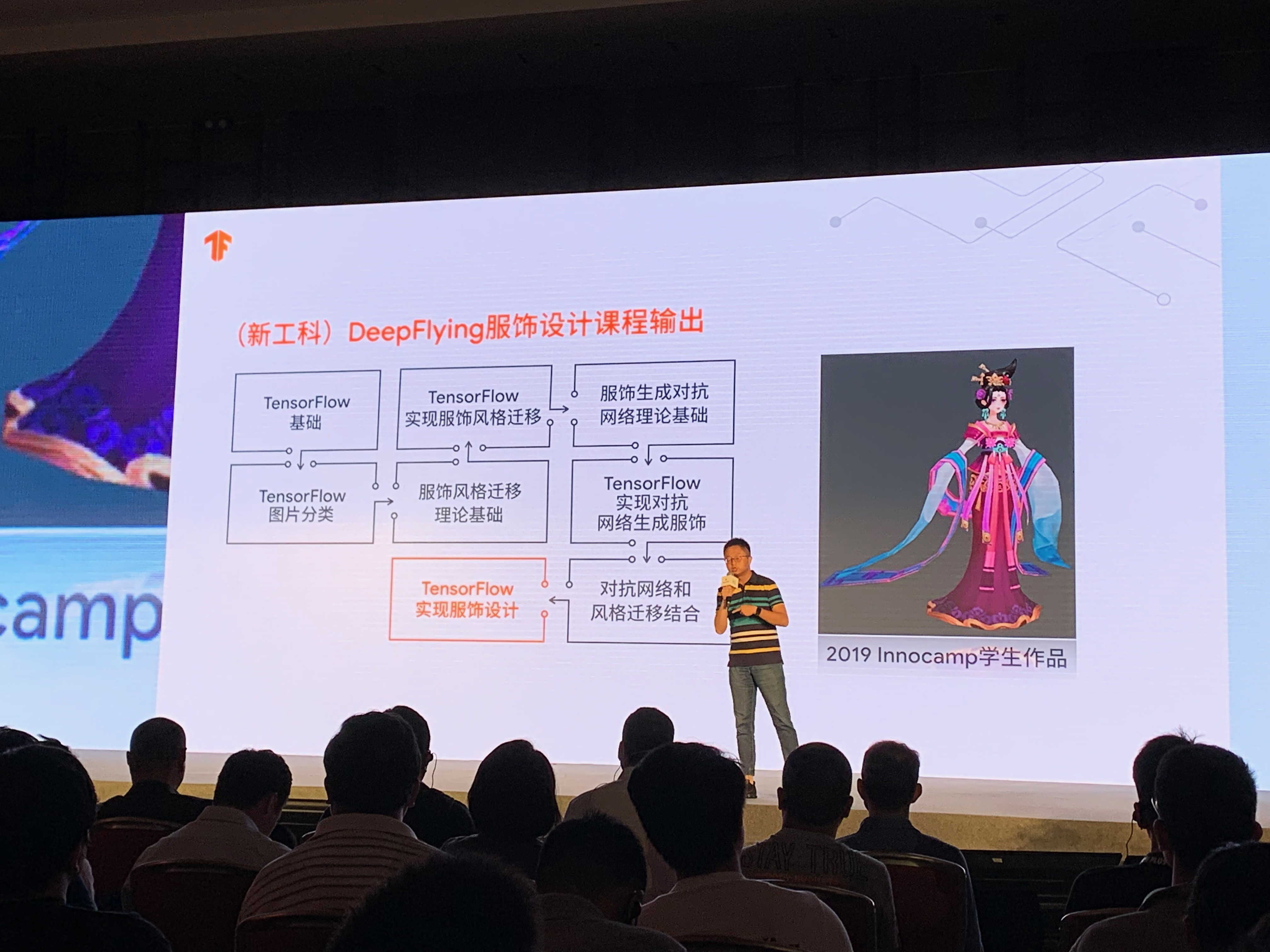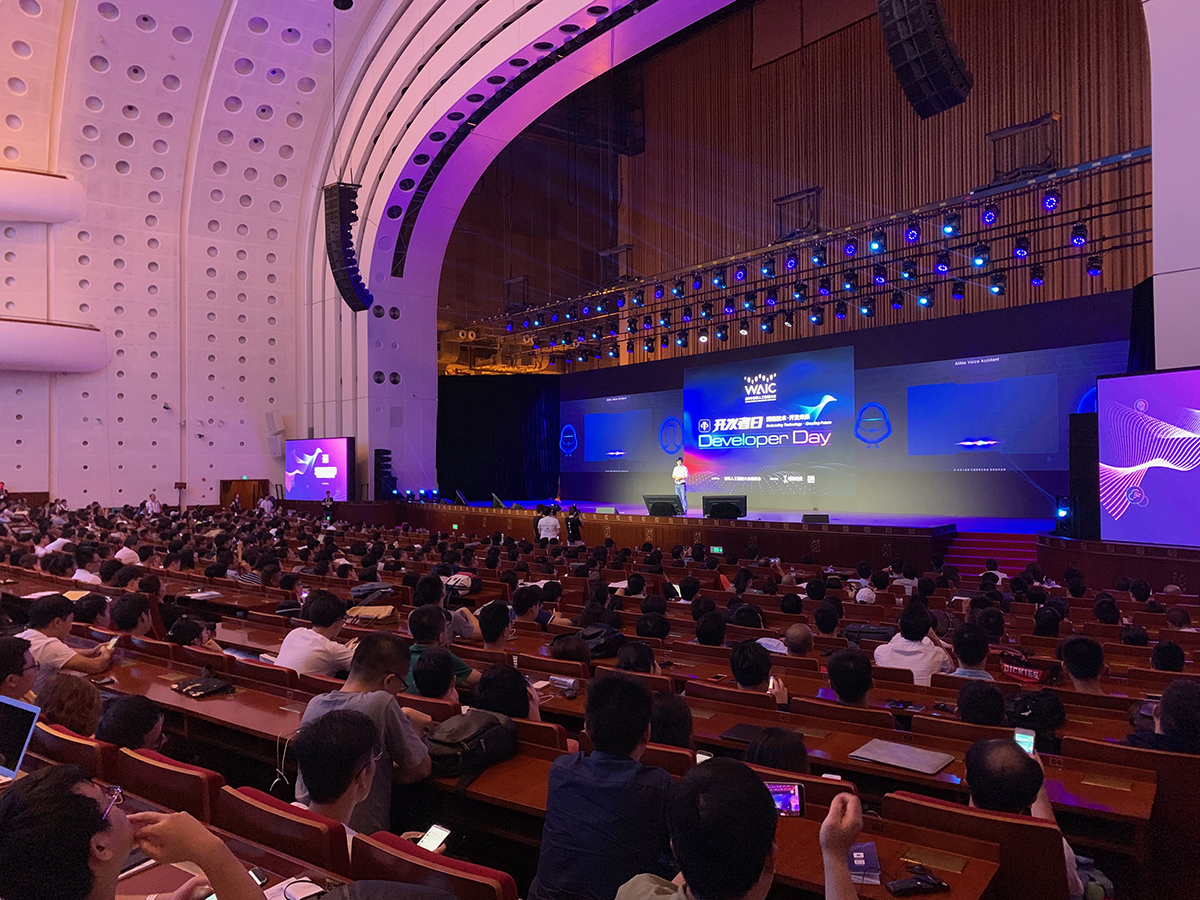(相比上海，最先发布各种关于人工智能政策文件的山东，一些东西还仍然在政策文件上，作为家乡人真的很为山东着急啊！)## 深度学习的冬天：是噱头 还是危机信号？]]>
<![CDATA[数据挖掘大赛内部赛赛后总结(上)]]> 2019-08-18T23:54:35+08:00 https://zning.me/15661436758435.html 最近参与了单位内举办的数据挖掘大赛内部赛，参与后感触良多，现就此进行简单总结。总结分为两篇：一篇为经验与经历总结，另一篇偏向于挖掘中真实技术技巧的。本篇为第一篇，经验与经历总结。

## 比赛经验与教训

1. 特征工程一定要做好，否则后面的再多的模型上分也难。特征工程犹如地基一样，牢固则历经风雨，不牢则风雨飘摇。
2. 分类问题、回归问题等监督学习类问题一定要做好本地模型训练后的交叉验证，这样可以在本地就能判断训练情况的好坏。本地交叉验证不仅可以提高准确度，减少不必要的提交次数，也可以增加自己对模型的信心与认知度。
3. 无论如何不能怀疑自己已经建立好的有效的交叉验证结果。本地有效的交叉验证一旦建立完成，一切的一切都以本地交叉验证结果为基准，其升降代表了训练结果的升降，毋庸置疑。虽然可能本地交叉验证与远程提交的交叉验证结果有些许差距，但是这样的差距也是线性相关的，因此本地交叉验证仍然是有效的。
4. 对于远端排行榜上的分数应该有一个清醒的认识，尤其是A榜，分高不一定真高，有可能是过拟合。对A榜过拟合虽然是可以的，但是一定要自己清醒的认识到过拟合的情况是存在的，并在A榜就给出相关对策例如调整模型训练参数、调整优化特征工程、添加新特征、增加模型融合等方法来解决这些问题。同时应给自己心里对B榜或者Private可能会出现的分数差距做准备。
5. 榜单出现黑马不要自乱阵脚，一定要对自己的建模思路有一定的信心和定力。机器智能类比赛榜单不同于ACM榜单那样精准性极高，也不同于数学建模那种事后评分的情况。榜单只是给予一个人追赶目标的方向，如果建模思路正确、特征工程充足，按部就班来也是没问题的。当然出现黑马也可以激发自己去想是否有自己以为做的很好但是仍然有改进的地方。
6. 模型融合或单一模型有可能都能取得高分，需要看实际情况适时处理。本次比赛涉及到的均为实际业务上的问题，因此在没有高超的自动化特征工程的能力的情况下的话，自动特征工程+根据业务特性手工挖掘特征工程才是应该走的正途。而这样扎实的特征工程情况下，对模型是否做融合，需要自己根据比赛时间和参赛队伍的实际情况做判断。一般来说模型融合的效果要比单一模型要好，毕竟多模型融合对于问题的泛化能力一定是提高的，但是如果确实没有时间的情况下，应该着重对某一或某几个模型进行重点攻克，这样才能够把握比赛重点。
7. 在特征工程无法通过流水线或者纯数学理论来做到完美的情况下，通过对题目业务本身去手工生成特征，尤其是多表间的特征生成，可能是极为关键的。这次比赛虽然特征工程做到了比绝大部分队伍好一点，但是表间特征这块是欠缺的，直观上感觉表间特征去创造的时候一定要贴合业务，但是由于我们队伍是纯技术队伍，没人懂得业务，因此从这些地方来看特征工程做的并不完美。
8. 在A榜的时候，每个人都应该去做一个模型，同时在榜上验证模型情况。无论模型是何种表现，在B榜时，只要是交叉验证有效的模型，都可以通过模型融合提升大模型泛化能力，以求B榜高分。这次我们在B榜遗憾跌出奖金区也是因为前期没能考虑模型泛化的情况，对模型融合前的模型准备十分不足导致B榜没有时间去做模型的融合。当然，模型也不是越多越好，基本上一个三人队伍一个人专攻一种模型最后融合的结果才是最好的。
9. 调参要仔细！B榜关闭后，我们发现最后一天B榜提交的模型中有个参数有点问题，小改之后本地交叉验证有所提高……虽然具体情况无法在远端结果得知，但是仍然是有一线希望的。当然这与上一条的教训息息相关了。因为时间不够，精神紧绷的情况下就有些地方注意不到了。所以还是要吸取上面的教训，把前期工作做好。

## 未来展望

]]>
<![CDATA[Python安装第三方库/包的一些操作]]> 2019-08-12T22:19:46+08:00 https://zning.me/15656195860013.html 最近Python装包比较频繁，又碰上一些特殊情况，现就此进行简单总结。

## 常规联网安装

pip install XXX
#XXX为包名


## 离线安装(.whl)

pip install /where/the/file/path/XXX.whl
#XXX为.whl文件名，路径为绝对路径或./的相对路径，若不带路径则为当前目录


## .egg文件

egg文件是一种打包，后缀名可以改成rar，直接解压缩，按照源码的形式安装。

]]>
<![CDATA[Docker操作小结及GDG Docker第一次活动记录]]> 2019-08-12T21:52:43+08:00 https://zning.me/15656179630389.html 最近有些地方需要Docker的使用，为了总结近期经验并且重拾Docker相关技术以及GDG Docker第一次活动记录，对一些操作进行重新总结。

## Docker常用操作

docker images


docker ps -a


docker logs 容器名或容器id


docker kill $(docker ps -a -q)  删除所有已经停止的容器： docker rm$(docker ps -a -q)


docker rmi $(docker images -q -f dangling=true)  删除所有镜像： docker rmi$(docker images -q)


docker search mysql


docker pull [REGISTRYHOST/][USERNAME/]NAME[:TAG]


sudo docker commit <CONTAINER ID> imagename01


sudo docker export <CONTAINER ID> > /home/export.tar


sudo docker save imagename01 > /home/save.tar


cat /home/export.tar | sudo docker import - imagename02:latest
save.tar文件转成镜像：

docker load < /home/save.tar


sudo docker images


## GDG Docker第一次活动记录

### 查看Docker 版本

docker -–version #版本信息
docker info #配置信息
docker -–help #帮助信息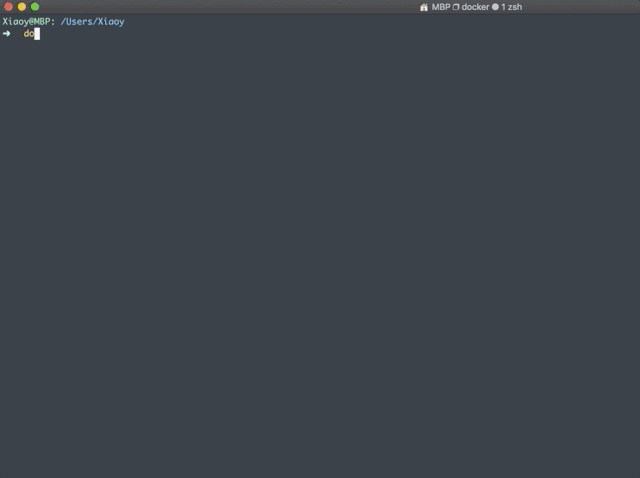### 运行你的第一个docker 镜像

docker run hello-world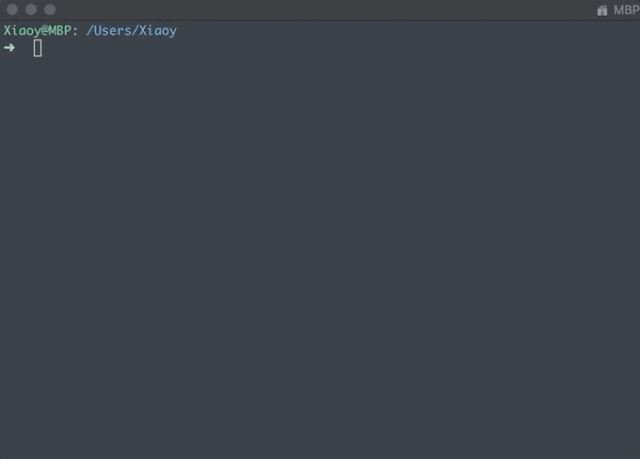### Docker 命令行工具介绍

Docker CLI 指令分为两类，一类是 Management Commands，一类是镜像与容器Commands。

Docker 的命令格式为：

docker [OPTIONS] COMMAND


docker pull :  从镜像仓库中拉取镜像

docker pull Ubuntu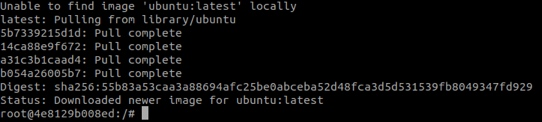docker run :

docker run –it ubuntu:latest

• -i : interactive mode
• -t: 分配tty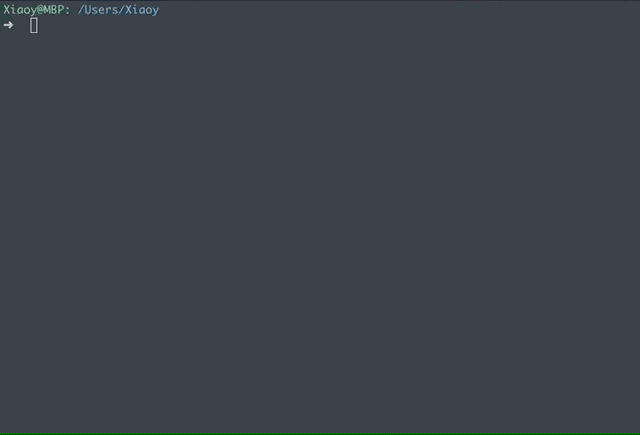Docker-CLI 与Linux 语法具有相似性，例如：

docker image ls
docker container ls
docker container ps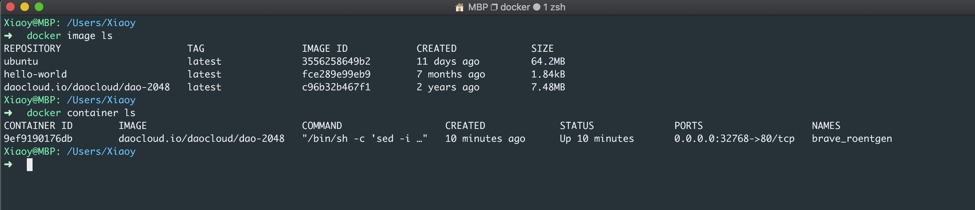#### 实战-1：2048 Web

docker run -d -P daocloud.io/daocloud/dao-2048


Tip: -d 表示容器启动后在后台运行

docker ps 查看容器运行状态如图：### Dockerfile 简介

Docker 可以从Dockerfile 文件中构建镜像，Dockerfile 语法请参考：

https://docs.docker.com/engine/reference/builder/

FROM : 这会从 Docker Hub 中拉取镜像，目的镜像基于所拉取的镜像进行搭建

WORKDIR: RUNCMDENTRYPOINTCOPY, ADD以此为工作路径

COPY：拷贝文件或文件夹到指定路径

RUN：镜像的最上层执行命令，执行后的结果会被提交，作为后续操作基于的镜像。

EXPOSE：暴露端口号

ENV：设置环境变量

CMD["executable","param1","param2"]：一个Dockerfile 应该只有一处 CMD 命令，如果有多处，则最后一处有效。

#### 实战 -2：搭建Python Web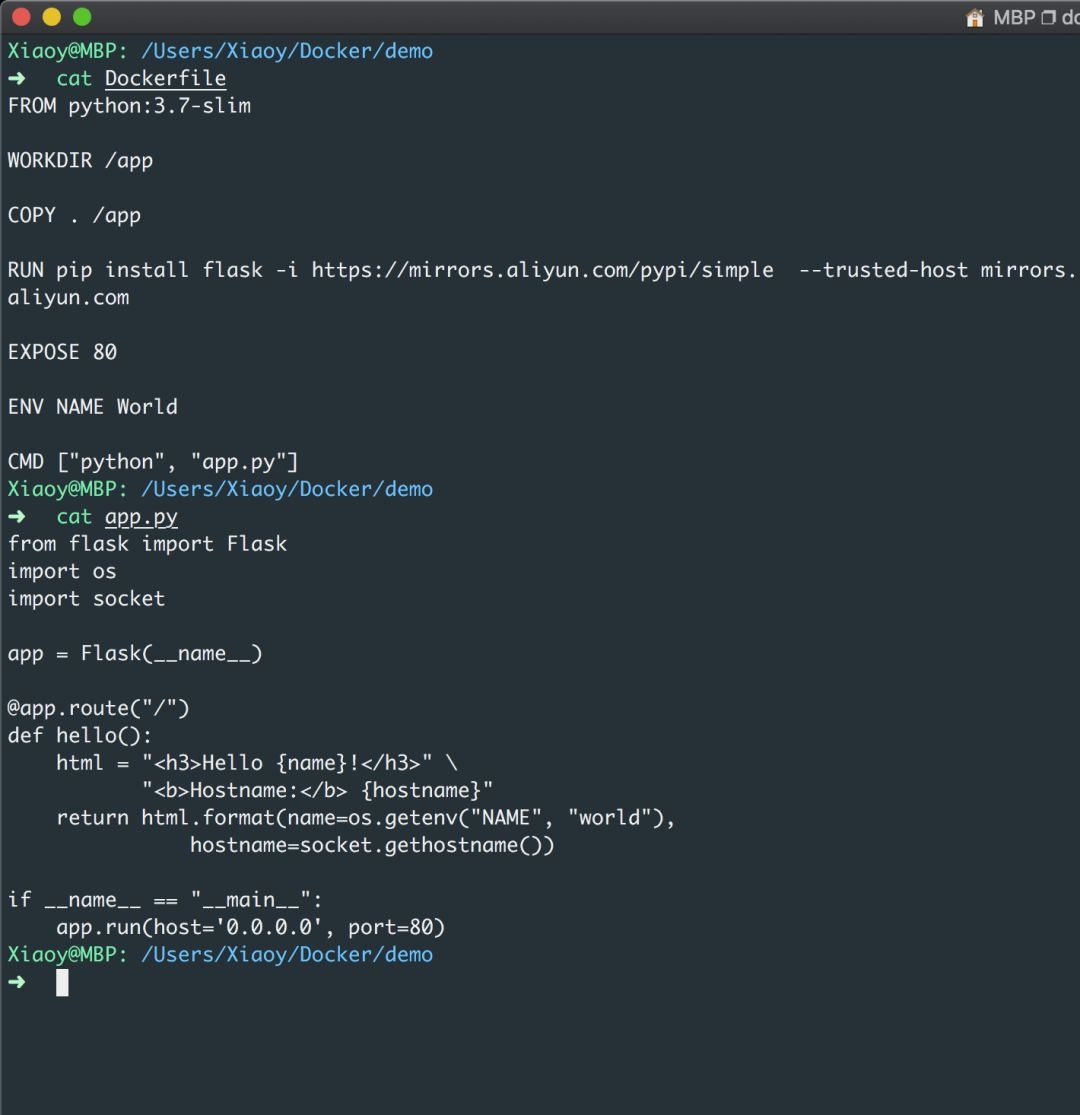docker build --tag=friendlyhello . #build
docker run -p 4000:80 friendlyhello #run
curl http://localhost:4000 #test
docker container stop XXX #stop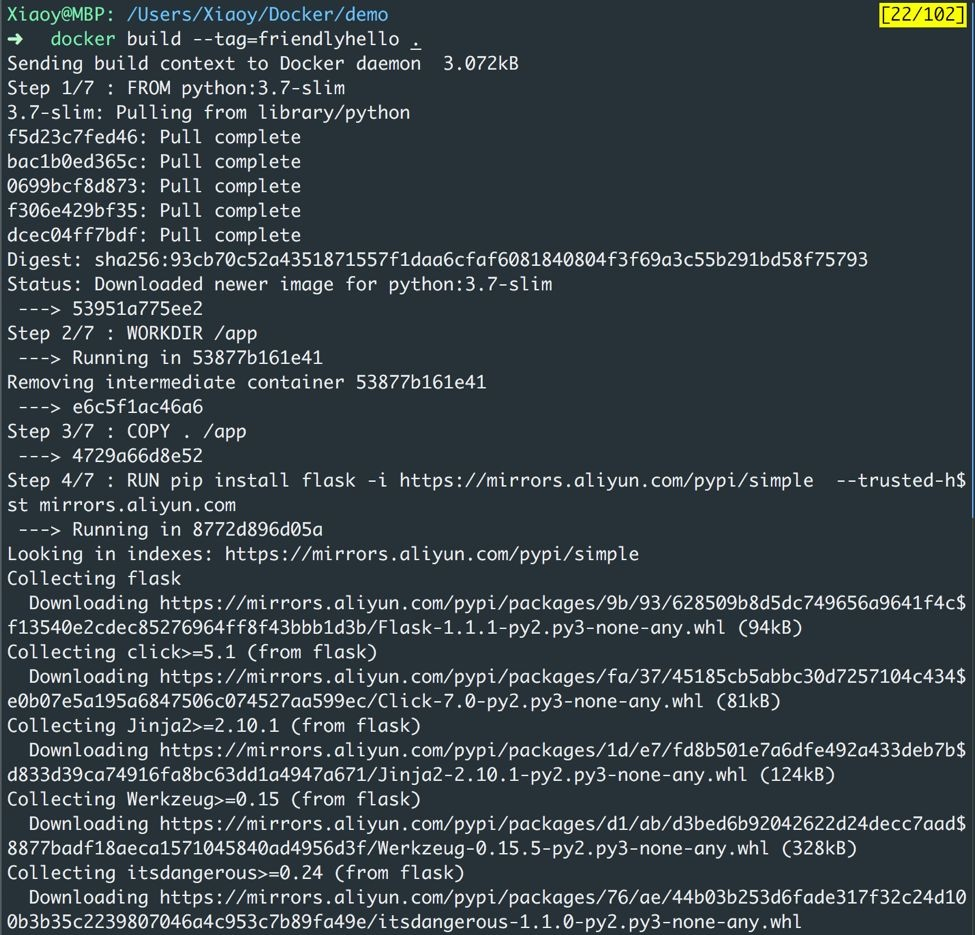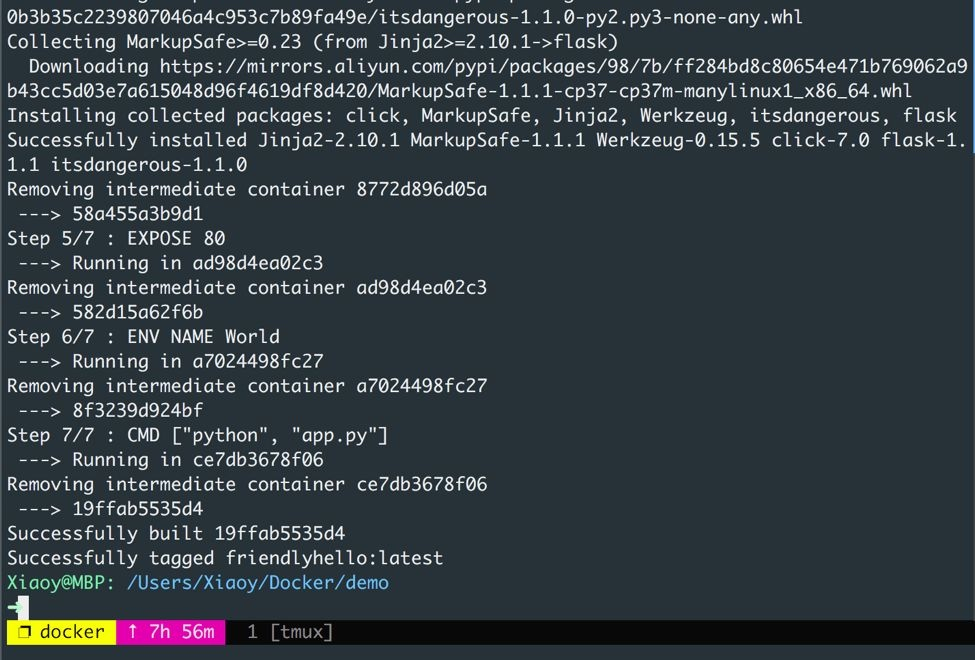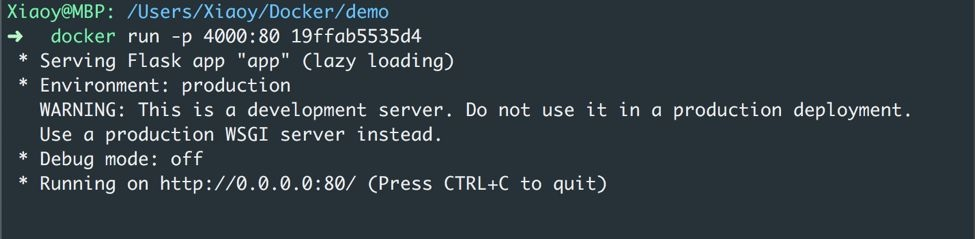Docker 生成container时会生成一个唯一的container-id，在上图中stop 命令用到了container-id。当然，你可以使用docker tag 命令对container 进行重命名。

Tip:

-p 4000:80 : 指的是从宿主机端口4000 映射到容器端口80## 参考文献

]]>
<![CDATA[深度学习之《深度学习入门》学习笔记（三）神经网络]]> 2019-08-12T21:46:04+08:00 https://zning.me/15656175642754.html 最近学习吴恩达《Machine Learning》课程以及《深度学习入门：基于Python的理论与实现》书，一些东西总结了下。现就后者学习进行笔记总结。本文是本书的学习笔记（三）神经网络。

1. 即便对于复杂的函数，感知机也隐含着能够表示它的可能性。
2. 但设定权重的工作，即确定合适的、能符合预期的输入与输出的权重，现在还是由人工进行的。

## 激活函数

### sigmoid函数

$h(x)=\frac{1}{1+exp(-x)}$

import numpy as np
import matplotlib.pylab as plt
#实现简单的阶跃函数
def step_function0(x):
if x > 0:
return 1
else:
return 0

def step_function1(x):
y = x >0
return y.astype(np.int)

def step_function(x):
return np.array(x > 0, dtype=np.int)

x = np.arange(-5.0, 5.0, 0.1)
y = step_function(x)
plt.plot(x, y)
plt.ylim(-0.1, 1.1) # 指定y轴的范围
plt.show()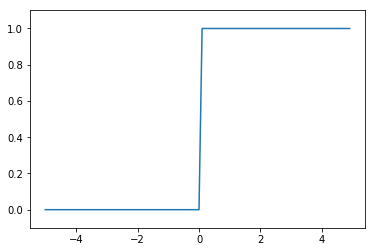#sigmoid函数
def sigmoid(x):
return 1 / (1 + np.exp(-x))

yy = sigmoid(x)
plt.plot(x,yy)
plt.ylim(-0.1,1.1)
plt.show()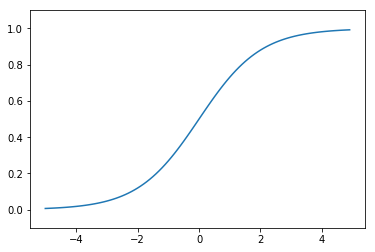plt.plot(x,y,linestyle = "--",label="step")
plt.plot(x,yy,label="sigmoid")
plt.show()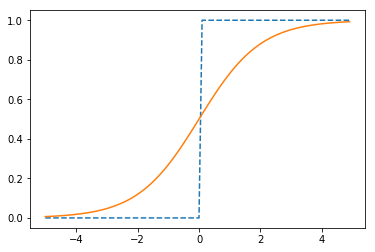1. sigmoid函数具有平滑性。
2. 两个函数结构均是“输入小时，输出接近0或为0，随着输入增大，输出趋向于1或变为1”，也就是说，当输入信号为重要信息时，阶跃函数和sigmoid函数都会输出较大的值；当输入信号为不重要的信息时，两者都输出较小的值。
3. 不管输入信号多大多小，输出信号值范围在0-1之间。
4. 两者均为非线性函数。

### ReLU函数

ReLU函数是一个非常简单的函数。ReLU函数在输入大于0时，直接输出该值；在输入小于0时，输出0。ReLU函数可以表示为下面的式子：

$h(x)=\begin{cases} x&(x>0) \\ 0&(x\leq 0) \end{cases}$

#ReLU函数实现
def relu(x):
return np.maximum(0,x)

y3 = relu(x)
plt.plot(x,y3)
plt.show()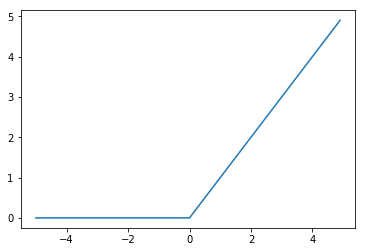## 多维数组的运算

# 一维数组
import numpy as np
A = np.array([1, 2, 3, 4])
print(A)


[1 2 3 4]

np.ndim(A)#获取数组维数


1

A.shape#获取数组的形状


(4,)

A.shape#其结果是个元组（tuple）


4

# 二维数组,也是矩阵
B = np.array([[1,2], [3,4], [5,6]])
print(B)


[[1 2]
[3 4]
[5 6]]

np.ndim(B)


2

B.shape


(3, 2)

# 矩阵乘法
C = np.array([[1,2],[3,4]])
C.shape


(2, 2)

D = np.array([[5,6],[7,8]])
D.shape


(2, 2)

np.dot(C,D)#乘积为点积


array([[19, 22],
[43, 50]])

np.dot(D,C)#绝大部分矩阵不满足乘法交换律


array([[23, 34],
[31, 46]])

### 神经网络的内积

# 使用NumPy矩阵实现神经网络
X=np.array([1,2])
X.shape


(2,)

W=np.array([[1,3,5],[2,4,6]])
print(W)


[[1 3 5]
[2 4 6]]

W.shape


(2, 3)

YY=np.dot(X,W)
print(YY)


[ 5 11 17]

## 3层神经网络的实现

# 多维数组实现A(1)=XW(1)+B
# 输入层到第1层的信号传递
X=np.array([1.0, 0.5])
W1=np.array([[0.1,0.3,0.5],[0.2,0.4,0.6]])
B1=np.array([0.1,0.2,0.3])

print(W1.shape)
print(X.shape)
print(B1.shape)


(2, 3)
(2,)
(3,)

A1=np.dot(X,W1)+B1
#观察第1层中激活函数的计算过程,激活函数使用sigmoid
Z1=sigmoid(A1)
print(A1)
print(Z1)


[0.3 0.7 1.1]
[0.57444252 0.66818777 0.75026011]

#第1层到第2层的信号传递
W2=np.array([[0.1,0.4],[0.2,0.5],[0.3,0.6]])
B2=np.array([0.1,0.2])

print(Z1.shape)
print(W2.shape)
print(B2.shape)
A2=np.dot(Z1,W2) + B2
Z2=sigmoid(A2)


(3,)
(3, 2)
(2,)

#第2层到输出层的信号传递
#定义输出层的激活函数为恒等函数
def identity_function(x):
return x

W3=np.array([[0.1,0.3],[0.2,0.4]])
B3=np.array([0.1,0.2])
A3=np.dot(Z2,W3)+B3
Y=identity_function(A3)
print(Y)


[0.31682708 0.69627909]

### 代码实现小结

# 3层网络实现案例，把权重记为大写字母W1，其他的偏置或中间结果等用小写字母表示
def init_network():
network = {}
network['W1'] = np.array([[0.1,0.3,0.5],[0.2,0.4,0.6]])
network['b1'] = np.array([0.1,0.2,0.3])
network['W2'] = np.array([[0.1,0.4],[0.2,0.5],[0.3,0.6]])
network['b2'] = np.array([0.1, 0.2])
network['W3'] = np.array([[0.1,0.3],[0.2,0.4]])
network['b3'] = np.array([0.1,0.2])

return network

def forward(network, x):
W1, W2, W3 = network['W1'], network['W2'], network['W3']
b1, b2, b3 = network['b1'], network['b2'], network['b3']

a1 = np.dot(x, W1) + b1
z1 = sigmoid(a1)
a2 = np.dot(z1, W2) + b2
z2 = sigmoid(a2)
a3 = np.dot(z2, W3) + b3
y = identity_function(a3)

return y

network = init_network()
x = np.array([1.0, 0.5])
y = forward(network, x)
print(y)


[0.31682708 0.69627909]

## 输出层的设计

### 恒等函数和softmax函数

$y_{k}=\frac{exp(a_{k})}{\sum_{i=1}^{n}exp(a_{i})}$

$$exp(x)=e^x$$。上式表示假设输出层共有$$m$$个神经元，计算第$$k$$个神经元的输出$$y_{k}$$。softmax函数的分子是输入信号$$a_{k}$$的指数函数，分母是所有输入信号的指数函数的和。

#实现softmax函数
a = np.array([0.3, 2.9, 4.0])
exp_a = np.exp(a) #指数函数
print(exp_a)
sum_exp_a = np.sum(exp_a) #指数函数的和
print(sum_exp_a)
y = exp_a / sum_exp_a
print(y)

[ 1.34985881 18.17414537 54.59815003]
74.1221542101633
[0.01821127 0.24519181 0.73659691]

# 定义softmax函数。供以后使用(初始版)
def softmax1(a):
exp_a = np.exp(a)
sum_exp_a = np.sum(exp_a)
y = exp_a / sum_exp_a

return y


### 实现softmax函数时的注意事项

softmax实现需要注意溢出问题，因为softmax函数内有$$exp(x)$$的指数运算，会使数值变得很大。因此，softmax函数的实现可以按如下式改进：

$y_{k}=\frac{exp(a_{k})}{\sum_{i=1}^{n}exp(a_{i})}=\frac{C exp(a_{k})}{C \sum_{i=1}^{n}exp(a_{i})}=\frac{exp(a_{k}+log C)}{\sum_{i=1}^{n}exp(a_{i}+log C)}=\frac{exp(a_{k}+C^{'})}{\sum_{i=1}^{n}exp(a_{i}+C^{'})}$

# 演示计算溢出情况与解决
a = np.array([1010, 1000, 990])
np.exp(a) / np.sum(np.exp(a)) #softmax,并未正确被计算，报错


/opt/conda/lib/python3.6/site-packages/ipykernel_launcher.py:3: RuntimeWarning: overflow encountered in exp
This is separate from the ipykernel package so we can avoid doing imports until
/opt/conda/lib/python3.6/site-packages/ipykernel_launcher.py:3: RuntimeWarning: invalid value encountered in true_divide
This is separate from the ipykernel package so we can avoid doing imports until

array([nan, nan, nan])

c = np.max(a) #1010
a - c
np.exp(a - c) / np.sum(np.exp(a - c))


array([9.99954600e-01, 4.53978686e-05, 2.06106005e-09])

# 定义softmax函数。供以后使用(正式用版)
def softmax(a):
c = np.max(a)
exp_a = np.exp(a - c)#溢出对策
sum_exp_a = np.sum(exp_a)
y = exp_a / sum_exp_a

return y


### softmax函数的特征

softmax函数的输出是0.0到1.0之间的实数。并且，softmax函数的输出值的总和是1.输出总和为1是softmax函数的一个重要特质，这个特质让softmax函数的输出解释为“概率”。通过softmax函数，我们可以用概率的（统计的）方法处理问题。

# 可解释为“概率”的softmax函数
a = np.array([0.3, 2.9, 4.0])
y = softmax(a)
print(y)
np.sum(y)


[0.01821127 0.24519181 0.73659691]

1.0

## 手写数字识别

# 代码暂略
import sys, os
print(sys.path.append(os.pardir))


None

]]>
<![CDATA[深度学习之《深度学习入门》学习笔记（二）感知机]]> 2019-08-12T21:44:59+08:00 https://zning.me/15656174993229.html 最近学习吴恩达《Machine Learning》课程以及《深度学习入门：基于Python的理论与实现》书，一些东西总结了下。现就后者学习进行笔记总结。本文是本书的学习笔记（二）感知机。

$y=\begin{cases} 0&(w_1x_1+w_2x_2\leq\theta) \\ 1&(w_1x_1+w_2x_2>\theta) \end{cases}$

## 感知机的实现

# 与门
def AND(x1, x2):
w1, w2, theta = 0.5, 0.5, 0.7
temp = x1*w1 + x2*w2
if temp <= theta:
return 0
elif temp > theta:
return 1

AND(0, 0)


0

AND(1,0)


0

AND(0,1)


0

AND(1,1)


1

## 导入权重和偏置

# NumPy实现感知机与权重与偏置
import numpy as np
x = np.array([0, 1])#输入
w = np.array([0.5, 0.5])#权重
b = -0.7#偏置
w*x


array([0. , 0.5])

np.sum(w*x)


0.5

np.sum(w*x)+b#大约为-0.2，由于浮点数小数造成运算误差


-0.19999999999999996

# 使用权重和偏置实现与门
def AND2(x1, x2):
x = np.array([x1, x2])#输入
w = np.array([0.5, 0.5])#权重
b = -0.7#偏置
tmp = np.sum(w*x) + b
if tmp <= 0:
return 0
else:
return 1

# 实现与非门
def NAND2(x1, x2):
x = np.array([x1, x2])#输入
w = np.array([-0.5, -0.5])#权重
b = 0.7#偏置
tmp = np.sum(w*x) + b
if tmp <= 0:
return 0
else:
return 1
# 实现或门
def OR2(x1, x2):
x = np.array([x1, x2])#输入
w = np.array([0.5, 0.5])#权重
b = -0.2#偏置
tmp = np.sum(w*x) + b
if tmp <= 0:
return 0
else:
return 1


## 多层感知机

# 异或门的实现
def XOR2(x1, x2):
s1 = NAND2(x1, x2)
s2 = OR2(x1, x2)
y = AND2(s1, s2)
return y

XOR2(0, 0)


0

XOR2(1, 0)


1

XOR2(0, 1)


1

XOR2(1,1)


0

1. 第0层的两个神经元接收输入信号，并将信号发送至第1层的神经元。
2. 第1层的神经元将信号发送至第2层的神经元，第2层的神经元输出$$y$$。

]]>